# Section 5`ggplot2` and the grammar of graphics

By Raphael Scherrer, data from Anne-Marie Veenstra-Skirl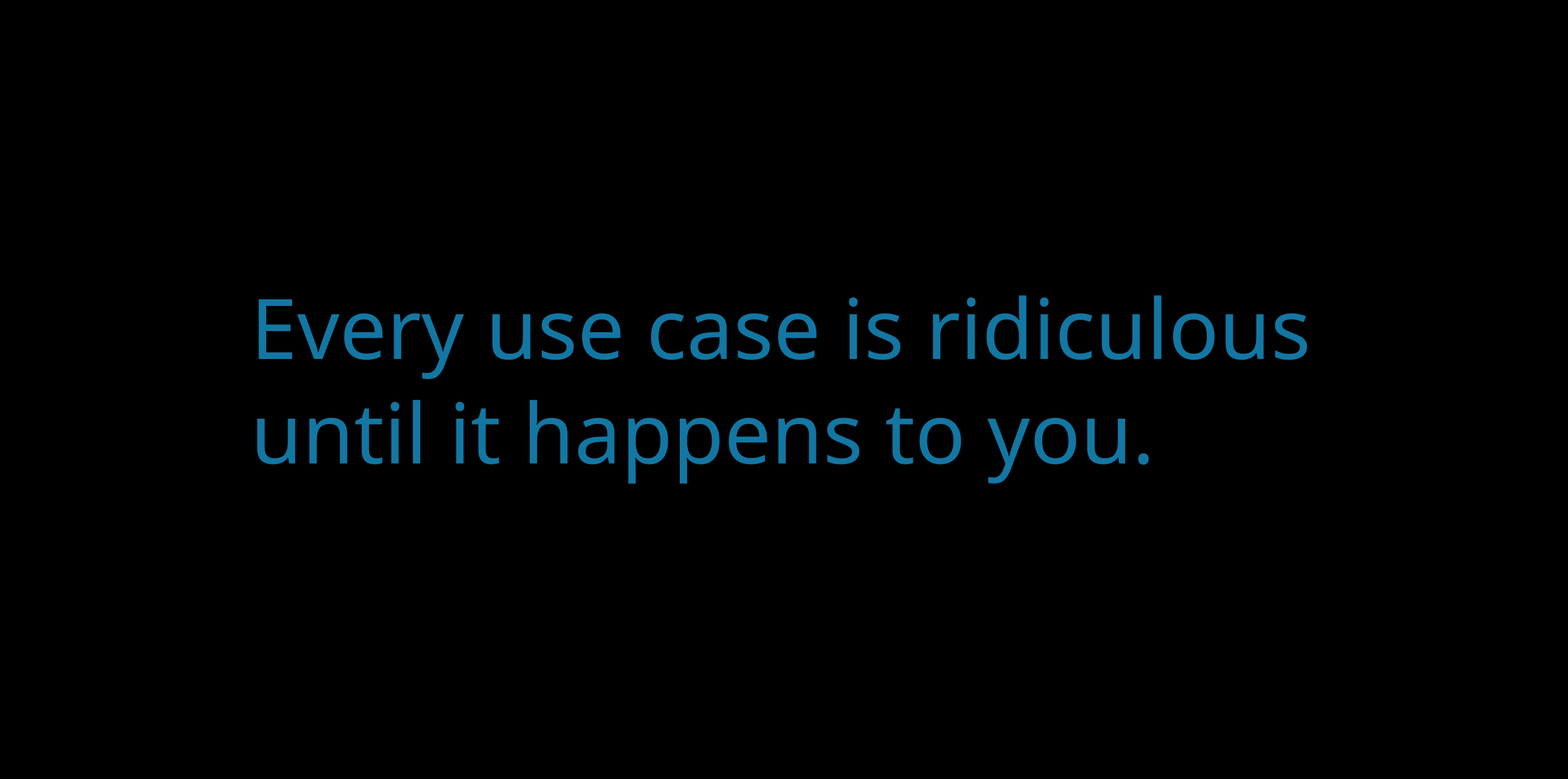In this tutorial we will learn how to make nice graphics using `ggplot2`, perhaps the most well-known member of the tidyverse. So well-known, in fact, that people often know `ggplot2` before they get to know about the tidyverse. We will first learn about the philosophy behind `ggplot2` and then follow that recipe to build more complex customized plots through some examples.

## 5.1 Introduction

### 5.1.1 What is `ggplot2` and why use it?

There are many ways of making graphics in base R. For example, `plot` is used for scatterplots, `hist` is used for histograms, `boxplot` is self-explanatory, and `image` can be used for heatmaps. However, those functions are often developed by different people with different logics in mind, which can make them inconsistent with each other, e.g. one has to learn what the arguments of each function are and switching from one type of visualization to another may not be very easy. `ggplot2` is aimed at solving this problem and making plotting flexible, allowing to build virtually any graph using a common standard, the grammar of graphics (which is what “gg” stands for). By building on a single reference grammar, `ggplot2` fits nicely into the tidyverse, and as part of it, it also follows the same rule as `tidyr`, `dplyr` or `purrr`, making the integration between all those packages very smooth.

### 5.1.2 What is the grammar of graphics?

The grammar of graphics is a system of rules on how to structure plots such that almost any graph can be made through combinations of a limited set of simpler elements, just as you can make any sentence by combining together letters from an alphabet. `ggplot2` is the implementation of this philosophy in R, and comes with a limited set of layers, that you can pick and combine into an impressive variety of graphics, all based on the same syntax. But what are those elements?

Here is the backbone of a ggplot statement (I will from now on use “ggplot” to refer to an object of class `ggplot`, the output of the `ggplot` function and the object that contains our graphic), taken from the book R for Data Science:

``````ggplot(data = <DATA>) +
<GEOM_FUNCTION>(
mapping = aes(<MAPPINGS>),
stat = <STAT>,
position = <POSITION>
) +
<COORDINATE_FUNCTION> +
<FACET_FUNCTION>``````

This pseudocode snippet illustrates a fundamental aspect of `ggplot2`, which is that plots are built by successive commands, each corresponding to a layer, assembled together using the `+` operator. This might seem less practical than having a whole plot made in a single call to the `plot` function, but it is this modularity that actually gives `ggplot2` its flexibility.

This means that in `ggplot2` you will typically need multiple commands to make a plot. All ggplots are made of at least the two following basic ingredients:

• A call to the `ggplot` function, with the relevant data frame passed to it (this data frame contains our data to plot)
• A `geom` layer, specifying the type of plot to be shown. Variables from the data are mapped onto the graphical properties of this layer, called aesthetics.

That means that:

``````library(tidyverse)
ggplot(mtcars)``````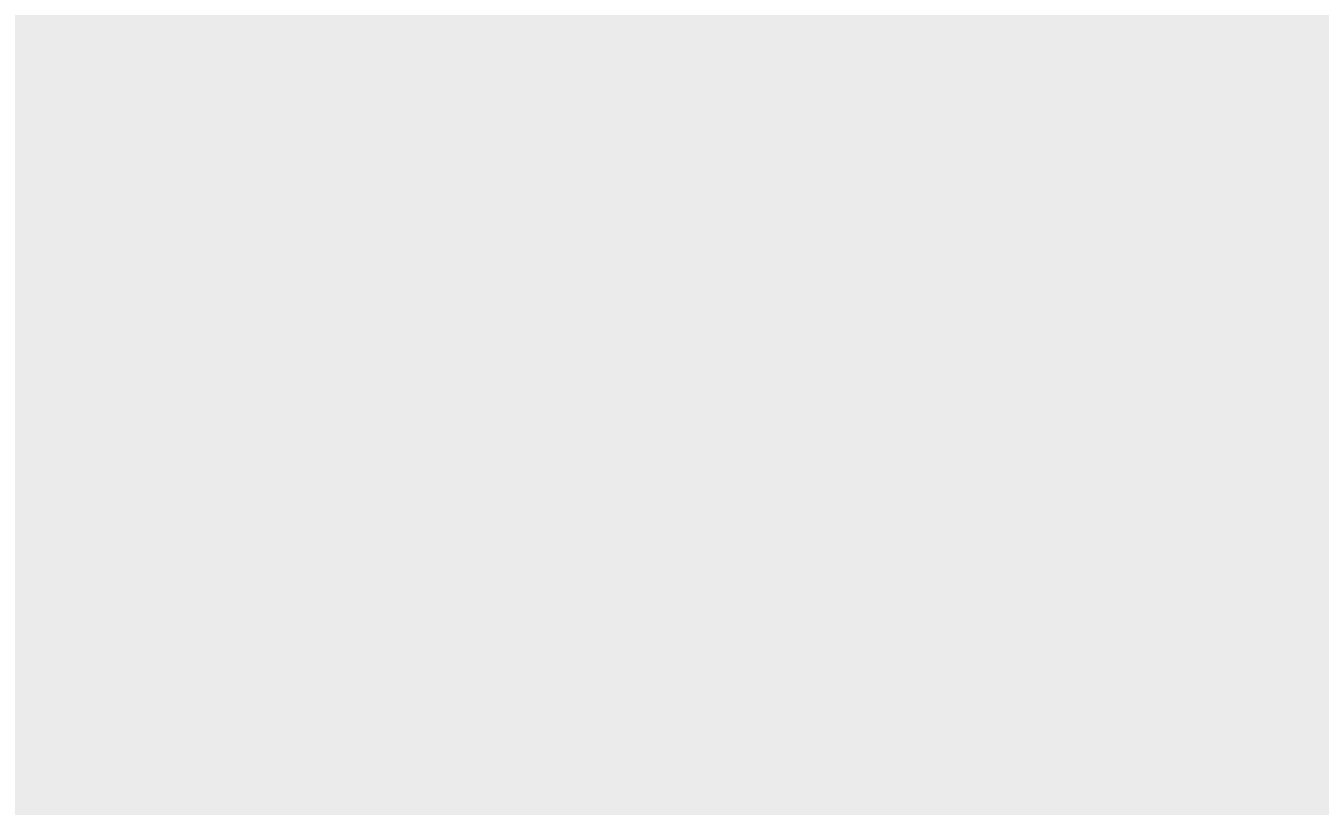will not show anything. A `ggplot` object is there, but it has no layers yet.

Plots can then be customized with statistical transformations, re-positioning, changes in coordinate system, facetting, and more. We will now go through the different elements.

### 5.1.3 Quick plot

Note that the `qplot` function, which stands for “quick plot”, will show a plot when called on your dataset. It is a wrapper around `ggplot2` layers that allows to quickly get a visualization, just like using `plot` from base R. However, it is less flexible than combining your ggplot yourself, so here we will make sure that you understand how the different layers are assembled.

## 5.2 But first, the data

In this chapter we will use the data from `bacterial_experiment.csv`, forged by Annie for us to use. This dataset resembles Annie’s experiment where she created mutator strains of bacteria (that is, bacteria that mutate at a much higher rate than usual) and tracked their growth through time and at different concentrations of an agent supposed to activate the full “mutation potential” of those strains.

``````data <- read_csv("data/bacterial_experiment.csv")
data
#> # A tibble: 310 x 7
#>   strain   assay   conc ratio time         cfu OD600
#>   <chr>    <chr>  <dbl> <dbl> <chr>      <dbl> <dbl>
#> 1 strain 1 test 1     1  8.58 T0     320000000 0.319
#> 2 strain 1 test 1     1  8.58 T1    1293846908 0.911
#> 3 strain 1 test 1     1  6.11 T0     370110830 0.287
#> 4 strain 1 test 1     1  6.11 T1    1480443320 0.9
#> 5 strain 1 test 1     1 11.8  T0     377928804 0.321
#> 6 strain 1 test 1     1 11.8  T1    1511715216 0.914
#> # … with 304 more rows``````

The different strains of bacteria were grown in two different `assay`s, whose details are irrelevant for the purpose of this tutorial. `cfu` is the number of colony forming units while `OD600` is the optical density at 600nm wavelength; both are estimates of bacterial population density. `ratio` represents the ratio in mutants between two time points, T0 and T1 (encoded in `time`).

In this table, the unit of observation is the time point (T0 and T1 are on different rows), therefore the values of `ratio`, which are attributed to each T0-T1 pair, are duplicated to yield one value per time point. To make our life easier with later plotting and to stay within the tidy spirit of the tidyverse (where one table should have one unit of observation), we use the tools we have already learnt to make a ratio-wise table:

``````data2 <- data %>%
pivot_wider(names_from = "time", values_from = c("cfu",  "OD600"))
data2
#> # A tibble: 155 x 8
#>   strain   assay   conc ratio    cfu_T0     cfu_T1 OD600_T0 OD600_T1
#>   <chr>    <chr>  <dbl> <dbl>     <dbl>      <dbl>    <dbl>    <dbl>
#> 1 strain 1 test 1     1  8.58 320000000 1293846908    0.319    0.911
#> 2 strain 1 test 1     1  6.11 370110830 1480443320    0.287    0.9
#> 3 strain 1 test 1     1 11.8  377928804 1511715216    0.321    0.914
#> 4 strain 1 test 1     1  7.78 369871771 1479487084    0.299    0.92
#> 5 strain 1 test 1     5 10.5  380000000 1505539596    0.295    0.922
#> 6 strain 1 test 1     5  8.29 322488344 1289953376    0.275    0.88
#> # … with 149 more rows``````

## 5.3 Geom layers

The `geom` object is the core visual layer of a plot, and it defines the type of plot being made, e.g. `geom_point` will add points, `geom_line` will add lines, etc. There are tons of geoms to pick from, depending on the type of figure you want to make, and new geoms are regularly added in extensions to `ggplot2` (links at the end of this chapter).

All geoms have aesthetics, or graphical parameters, that may be specified. Those include x and y coordinates, color, transparency, etc. Some aesthetics are mandatory for some geoms, e.g. `geom_point` needs `x` and `y` coordinates of the points to plot. Other aesthetics are optional, e.g. if `color` is unspecified, all the points will look black. Some geoms even have no mandatory aesthetics, such as `geom_abline`, which will plot a diagonal running through the origin and with slope one if its `intercept` and `slope` are unspecified.

Aesthetics are specified in two ways: (1) variables from the `data` can be mapped to them using the `aes` function, or (2) they can take fixed values.

Some of the main aesthetics to know, besides geom-specific coordinates (e.g. `x`, `y`), include: `color`, `fill` (color used to fill surfaces), `group` (used e.g. to plot multiple lines with similar aspect on the same plot), `alpha` (transparency), `size`, `linetype`, `shape`, and `label` (for showing text).

Note that in most functions across the tidyverse both US and UK English can be used, e.g. `colour` is also a valid aesthetics, and `dplyr::summarize` is equivalent to `dplyr::summarise`.

### 5.3.1 Mapping variables to aesthetics

Variables are mapped to aesthetics using the `aes` function. Here is a basic scatterplot example showing `ratio` against `conc`:

``````ggplot(data2) +
geom_point(mapping = aes(x = conc, y = ratio))``````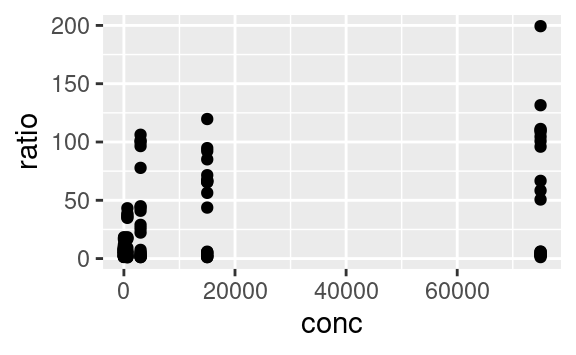We can use the other available aesthetics to show more aspects of the data, or to see patterns a bit more clearly. For example, we can color-code the points based on their strain, and change their shape based on the type of assay:

``````ggplot(data2) +
geom_point(mapping = aes(x = conc, y = ratio, color = strain, shape = assay))``````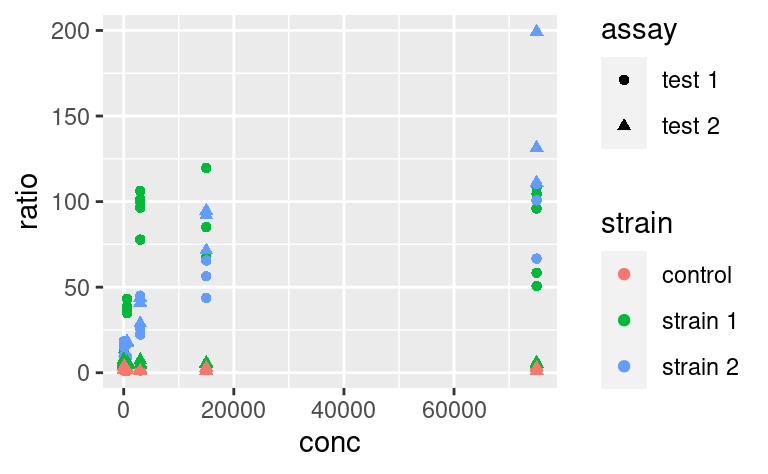Do you want to map several variables to a single aesthetic? Then `interaction` from base R can be used within a `ggplot`:

``````ggplot(data2) +
geom_point(
mapping = aes(x = conc, y = ratio, color = interaction(strain, assay))
)``````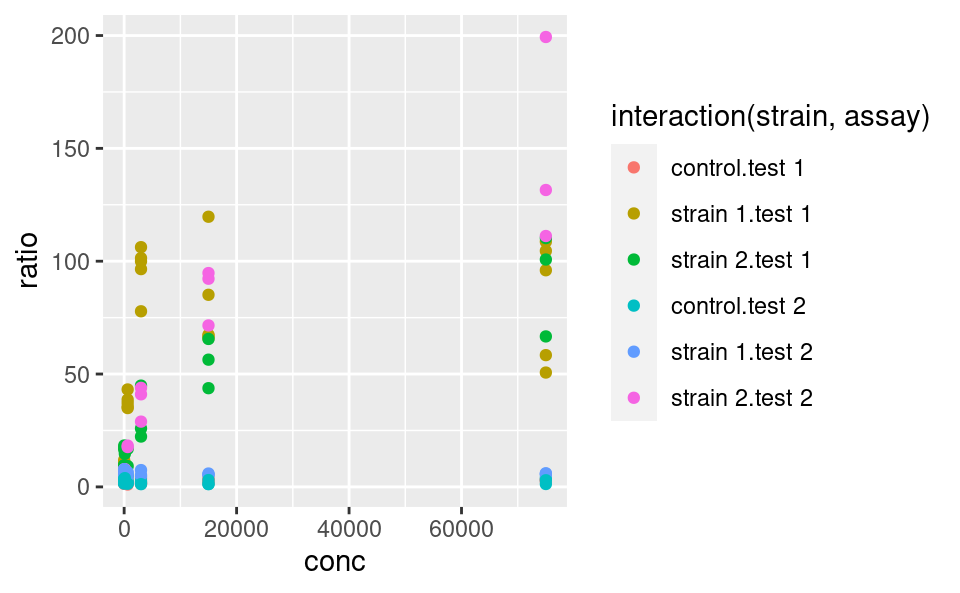### 5.3.2 Fixed aesthetics

Fixed graphical parameters (i.e. that are not mapped to a variable) should be added as arguments of the geom outside the `aes` command. For example, to make all points a little bigger and more transparent, we can use

``````ggplot(data2) +
geom_point(
mapping = aes(x = conc, y = ratio, color = strain, shape = assay),
size = 2, alpha = 0.6
)``````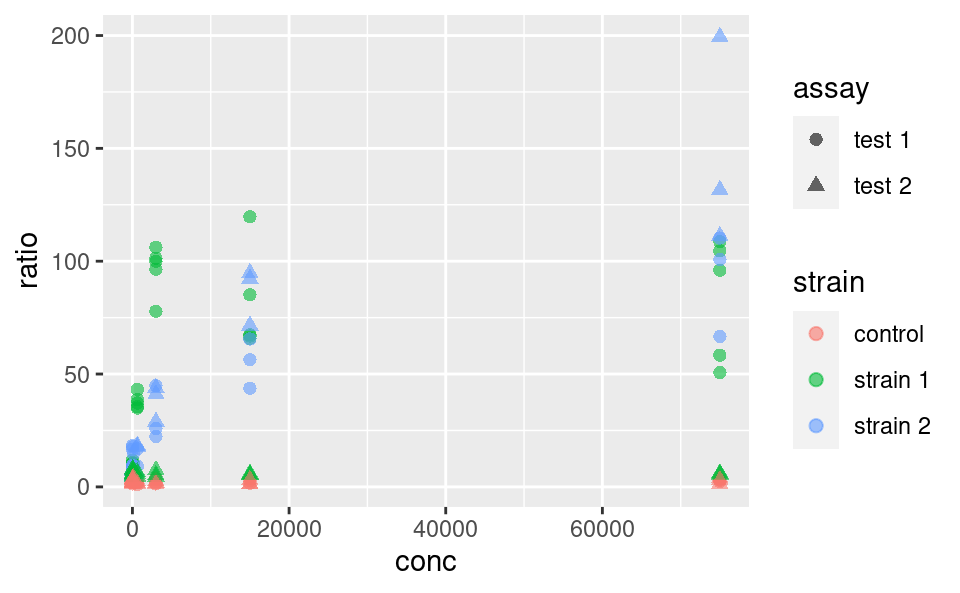### 5.3.3 Statistical transformation

Statistical transformations, or `stat` functions, can be applied to the data within a `geom` call. Actually, statistical transformations are always applied within a `geom` call, but most of the time the identity function is used. To illustrate, consider the following plot showing a distribution of `ratio` for different strains:

``````ggplot(data2) +
geom_density(aes(x = ratio, fill = strain), alpha = 0.5)``````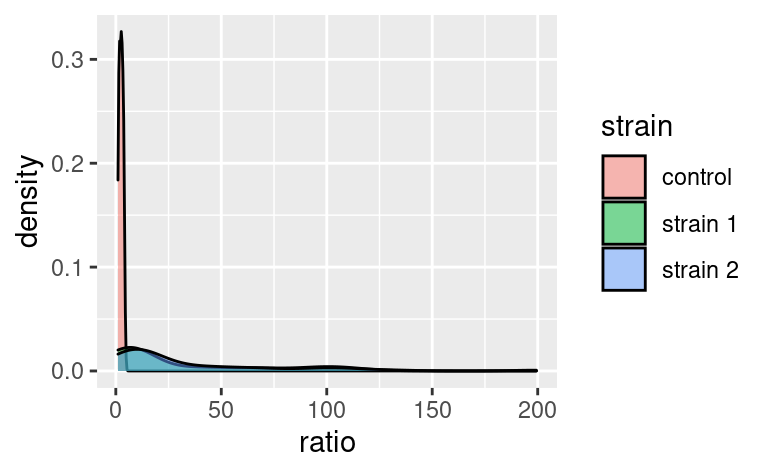Here, the `density` axis is not part of the original dataset `data2`; it was computed from the data, for each value of `ratio`, by using a density-estimation algorithm. This shows that `stat_density` (and not `stat_identity`) is the default `stat` used in `geom_density`. Every `geom` comes with its default `stat`.

Similarly, `stat` functions can be used in place of `geom` because every `stat` has a default `geom` associated to it. So, we can call:

``````ggplot(data2) +
stat_density(aes(x = ratio, fill = strain), alpha = 0.5)``````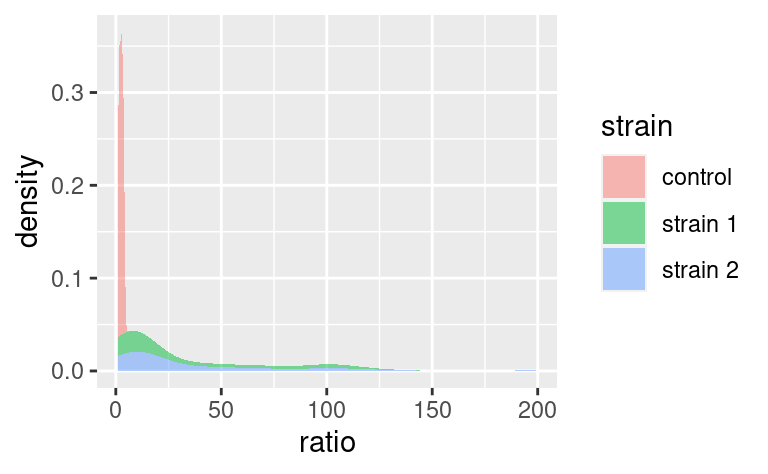which has `geom_density` as default `geom.`

It is possible to override the default `stat` using the `stat` argument of `geom`, and conversely, it is possible to change the default `geom` associated with a given `stat`. For example, say we want to plot our densities as points. Then,

``````ggplot(data2) +
stat_density(aes(x = ratio, color = strain), alpha = 0.5, geom = "point")``````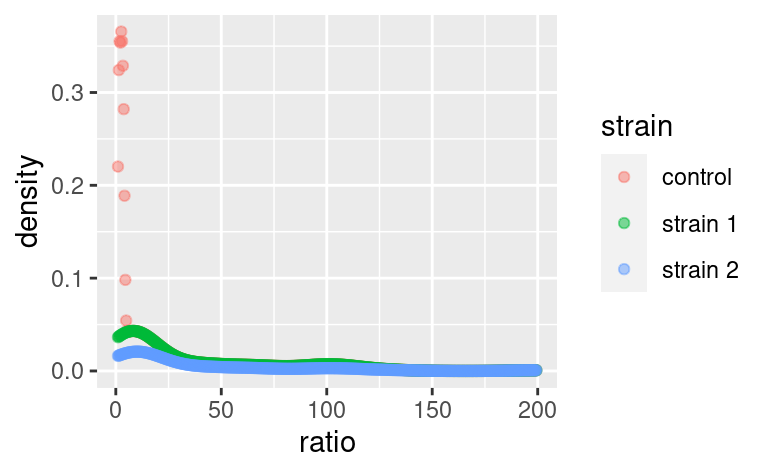does the job (note that we replaced `fill` with `color` because our points do not have a surface to fill).

Note that default `geom`-`stat` combinations are usually well thought of (density plots are a good example). Therefore, it is often not necessary to play with stats. It may matter in some specific cases, e.g. when using `geom_bar`, but we do not cover that here (you can check out the dedicated chapter in R for Data Science for an example).

### 5.3.4 Position

The `position` argument of geoms allows to adjust the positioning of the geom’s elements. It has a few variants, but the possibilities depend on the geom used. We illustrate those available to `geom_bar`. By default, `geom_bar` uses the `stat_count` statistical transformation, meaning that it will show us the number of observations into each category of a factor, e.g. `strain`, splitted into categories of another factor, e.g. `assay`:

``````ggplot(data2) +
geom_bar(aes(x = strain, fill = assay))``````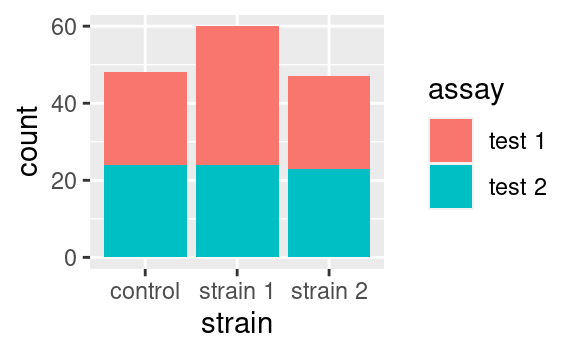If we wanted to visualize proportions instead of numbers, we could use the `fill` value of the `position` argument:

``````ggplot(data2) +
geom_bar(aes(x = strain, fill = assay), position = "fill")``````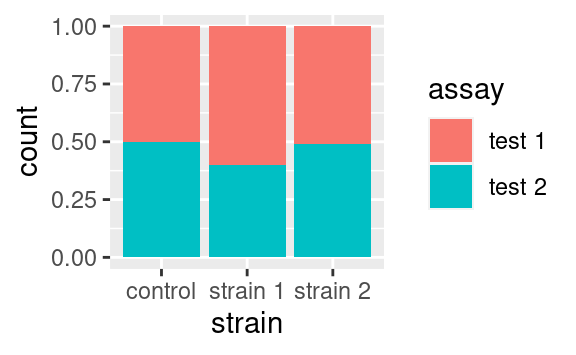Alternatively we could use the `dodge` option to show the different categories side-by-side:

``````ggplot(data2) +
geom_bar(aes(x = strain, fill = assay), position = "dodge")``````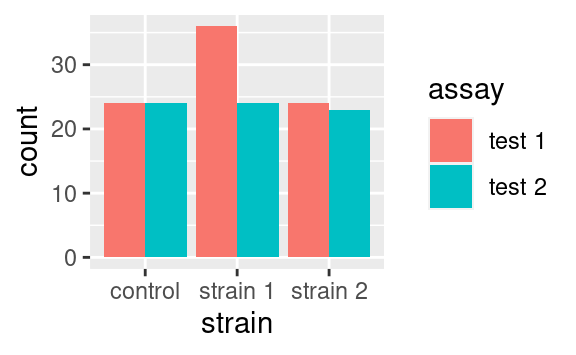Those are only two examples of what can be done. Just remember that `position` exists and look into the documentation of your geom of interest to see what position adjustments are available! (Check out `geom_jitter` as a nice wrapper around `geom_point` with a `jitter` position adjustment, perfect to overlay with boxplots or violin plots.)

### 5.3.5 Other geoms

The most common `geoms` you may encounter are:

• `geom_point` for scatter plots and `geom_jitter` for the dodged equivalent
• `geom_bar` for a barplot
• `geom_text` for a scatter plot of labels
• `geom_histogram` and `geom_density`, self-explanatory
• `geom_boxplot` and `geom_violin`
• `geom_line`, `geom_path` (a `line` never goes backwards along the x-axis, while a `path` can) and `geom_smooth` (local regression smoothing)
• `geom_segment`, `geom_hline`, `geom_vline` and `geom_abline` that may come handy as annotations
• `geom_tile` for heatmaps

There are litterally tons of geoms and ways to use them. In this tutorial, we emphasize the understanding of the grammar and how to assemble the different ingredients, rather than the ingredients themselves. For this reason, here we are not giving an exhaustive sample of each geom and what they look like. So, keep this list of names in mind as a reminder that whatever plot you want to make, there probably is a `geom` for it. To explore a gallery of examples, check out the R graph gallery.

### 5.3.6 Extra on aesthetics

It is possible to use the `+` operators, not only to add layers but also to modify previous layers. You might wonder why not to write the layer correctly in the first place. This starts making more sense in cases e.g. where a plot can be modified in different ways. For example, consider this plot:

``````ggplot(data2, aes(x = conc, y = ratio)) +
geom_point()``````We may want to color-code the points based on `strain` or `assay`, or both, thus requiring two plots building on this single one. An important property of `ggplot` objects is that they can be assigned to variables, e.g.

``````p <- ggplot(data2, aes(x = conc, y = ratio)) +
geom_point()``````

Note that we have to call the object `p` for the plot to be displayed. If we just assign the plot to `p`, the plot does not show. We can subsequently add differential aesthetics to different copies of `p`:

``````p + aes(color = strain)
p + aes(color = assay)``````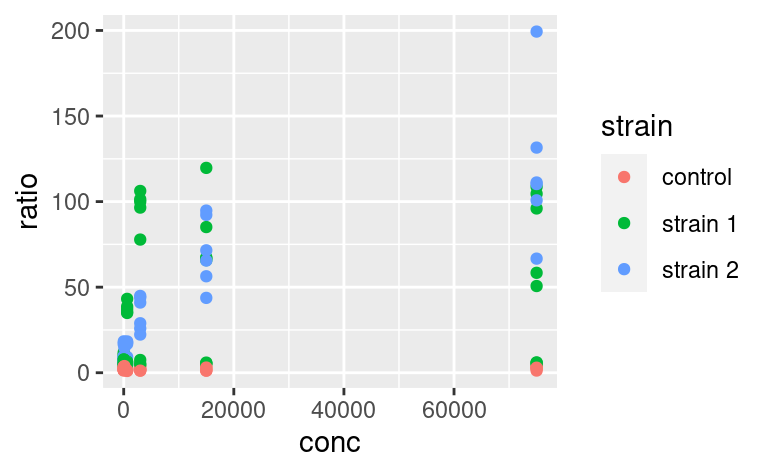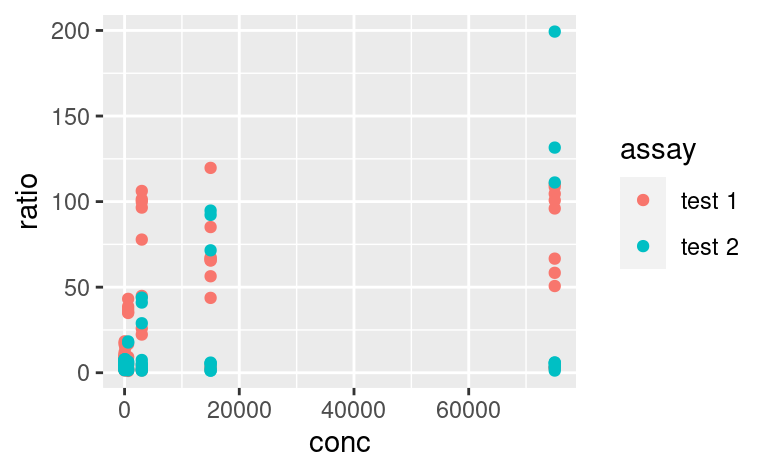### 5.3.7 Plot-wide aesthetics and multiple geoms

In the last example, by adding new aesthetics mapping to the ggplot using the `+` operator, we did not add these aesthetics specifically to the `geom_point` layer, but to all the geoms present in the plot. Similarly, one can pass aesthetic mappings to the `ggplot` command directly, not necessarily with the `geom` statement. This saves some typing when geoms taking the same aesthetics are used, e.g. `geom_violin` and `geom_jitter`:

``````ggplot(data2, aes(x = factor(conc), y = ratio)) +
geom_violin() +
geom_jitter(width = 0.1)
# x is made categorical here``````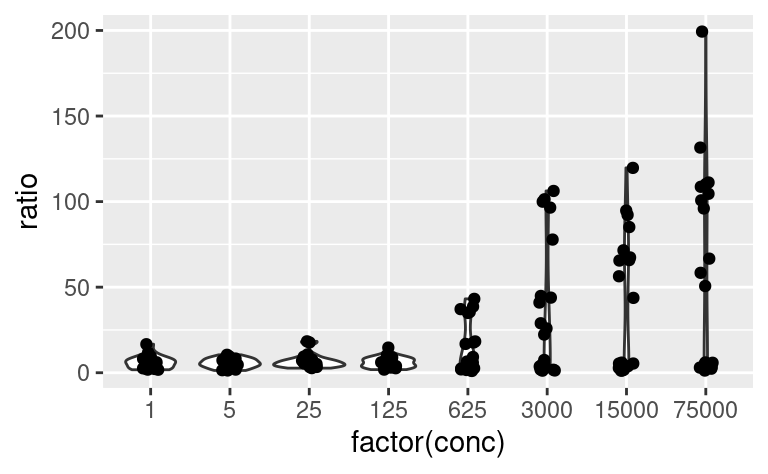This shows a nice example of multiple geoms combined in a single plot. If, however, the aesthetics used in some geoms are geom-specific, better pass them to their respective `geom`. For example, if you want to color only the points but not the violins, use:

``````ggplot(data2, aes(x = factor(conc), y = ratio)) +
geom_violin() +
geom_jitter(mapping = aes(color = strain), width = 0.2)``````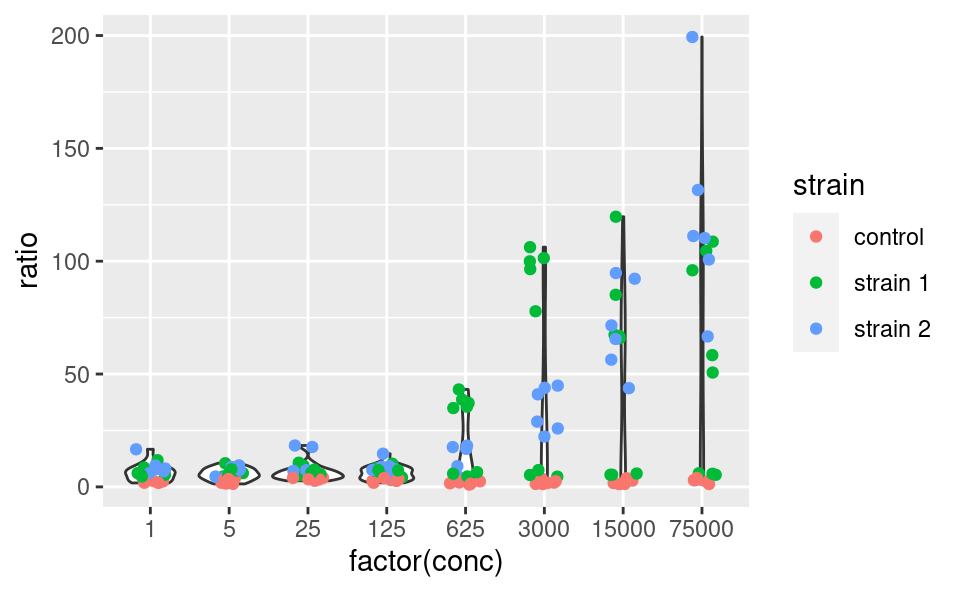### 5.3.8 Multiple geoms with different datasets

Just as aesthetics can vary from geom to geom, so do datasets. In other words, the dataset does not have to be passed to the `ggplot` command necessarily, and can be passed to a `geom` instead, for example:

``````ggplot() +
geom_point(data2, mapping = aes(x = conc, y = ratio, color = strain))``````This means that different geoms can be based on different datasets. This allows quite some complexification of the plots and illustrates very well the usefulness of the other packages of the tidyverse. Say, for example, that we want to add to this plot a line going through the means at each value of `conc`. These mean values are not yet present in our dataset, and we need to come up with a mean-wise dataset. `dplyr` is our friend for this task:

``````data3 <- data2 %>%
group_by(conc, strain) %>%
summarize(ratio = mean(ratio))
data3
#> # A tibble: 24 x 3
#> # Groups:   conc 
#>    conc strain   ratio
#>   <dbl> <chr>    <dbl>
#> 1     1 control   2.21
#> 2     1 strain 1  7.09
#> 3     1 strain 2  9.16
#> 4     5 control   2.50
#> 5     5 strain 1  7.17
#> 6     5 strain 2  6.89
#> # … with 18 more rows``````

Let us now add an extra layer of information based on this latest, summary dataset:

``````ggplot() +
geom_point(data = data2, mapping = aes(x = conc, y = ratio, color = strain)) +
geom_line(data = data3, mapping = aes(x = conc, y = ratio, color = strain))``````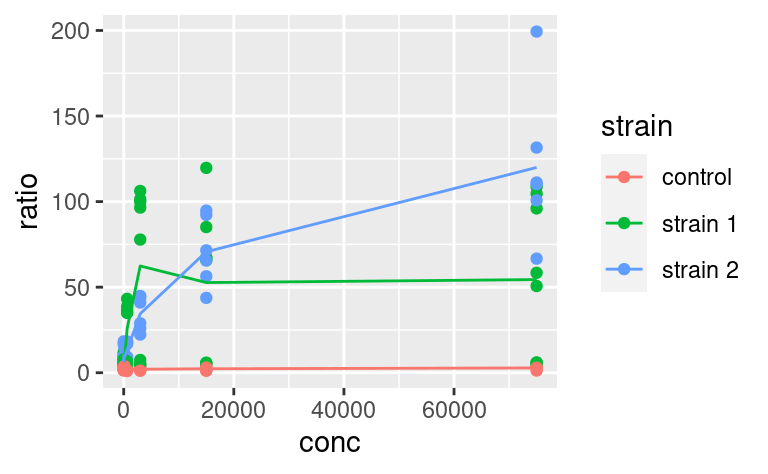Here, we could save some typing by writing:

`````` ggplot(data2, mapping = aes(x = conc, y = ratio, color = strain)) +
geom_point() +
geom_line(data = data3)``````where `geom_line` inherits the same aesthetic mapping as `geom_point`. But then, you have to make sure that `data3` contains all the aesthetics that the `ggplot` call expects to see in each of its `geoms` (here `x`, `y` and `color`).

## 5.4 Coordinate-system

The default way that the plotting window is organized is an orthogonal space with a horizontal x-axis and a vertical y-axis. Use the `coord` commands to deviate from this. For example, `coord_flip` will flip the axes:

``````ggplot(data2, aes(x = factor(conc), y = ratio)) +
geom_violin() +
coord_flip()``````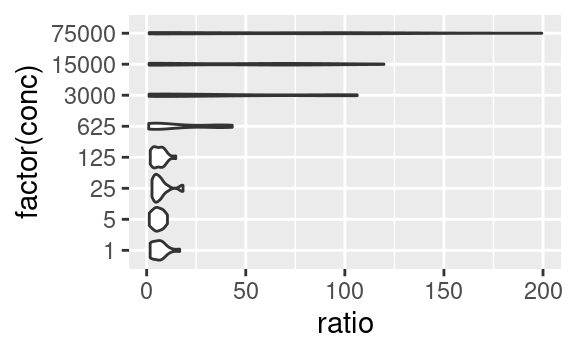while `coord_fixed` will fix the aspect ratio between the axes, thus showing them on the same scale. For example, the following plot of the optical density between two time points,

``````ggplot(data2, aes(x = OD600_T0, y = OD600_T1)) +
geom_point()``````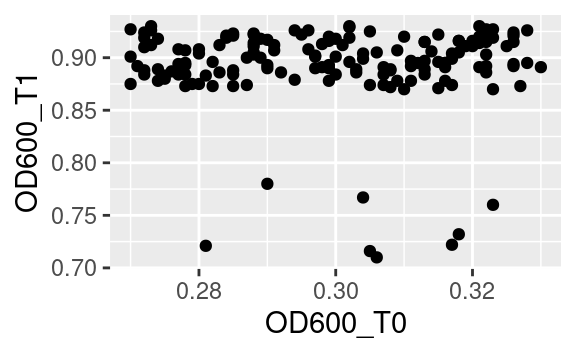becomes:

``````ggplot(data2, aes(x = OD600_T0, y = OD600_T1)) +
geom_point() +
coord_fixed()``````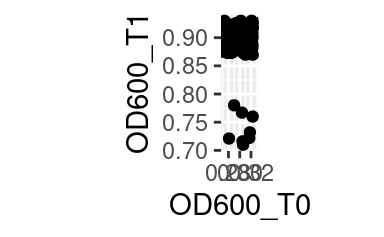when both axes are shown on the same scale.

Other coordinate systems exist, depending on the need, including `coord_polar` for radial plots or `coord_quickmap`, tailored at latitude-longitude plotting.

## 5.5 Facetting

One of the most powerful features of `ggplot2` is its easy way of splitting a plot into multiple subplots, or facets.

There are two functions for facetting: `facet_grid` and `facet_wrap`. `facet_grid` will arrange the plot in rows and columns depending on variables that the user defines:

``````ggplot(data2, aes(x = conc, y = ratio, color = strain)) +
geom_point() +
facet_grid(strain ~ assay)``````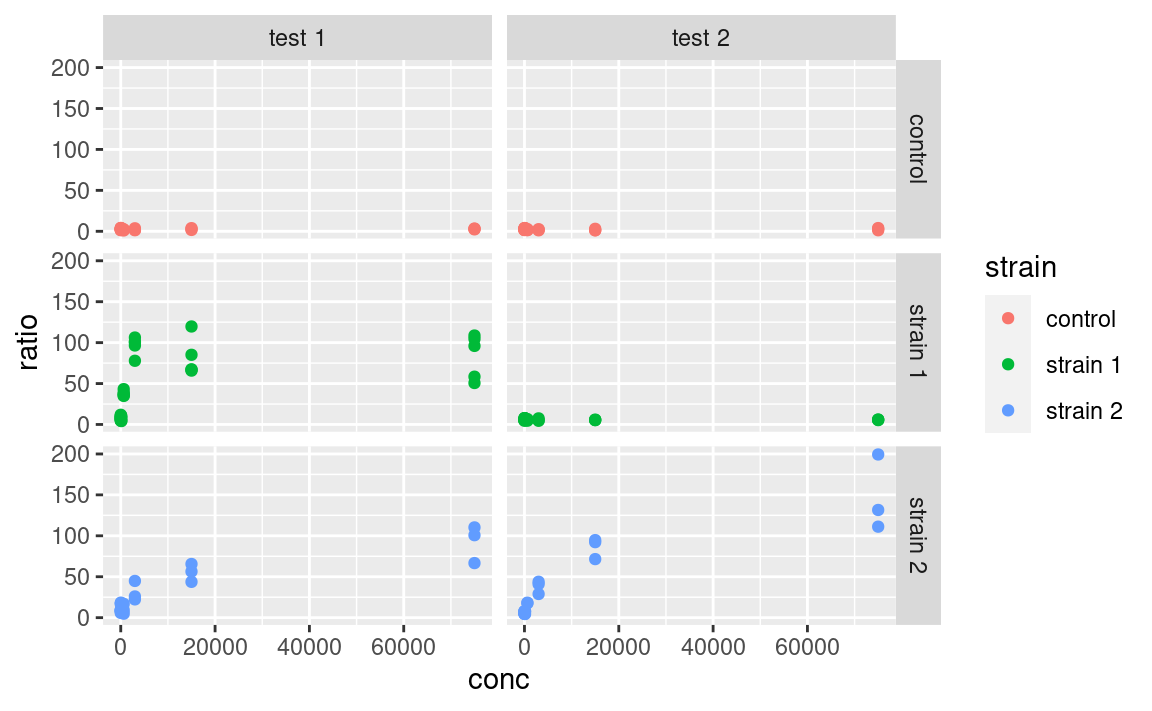Here the tilde (`~`) symbolizes a formula, a type of expression in R with a left and right-hand side, which here are interpreted as variables to use for rows and columns, respectively. If using only one variable for facetting, use `.` or nothing on the other side of the tilde.

Note that facets are plotted on the same scale. We can use the `scales` argument to allow free scales, for example:

``````ggplot(data2, aes(x = conc, y = ratio, color = strain)) +
geom_point() +
facet_grid(strain ~ assay, scales = "free_y")``````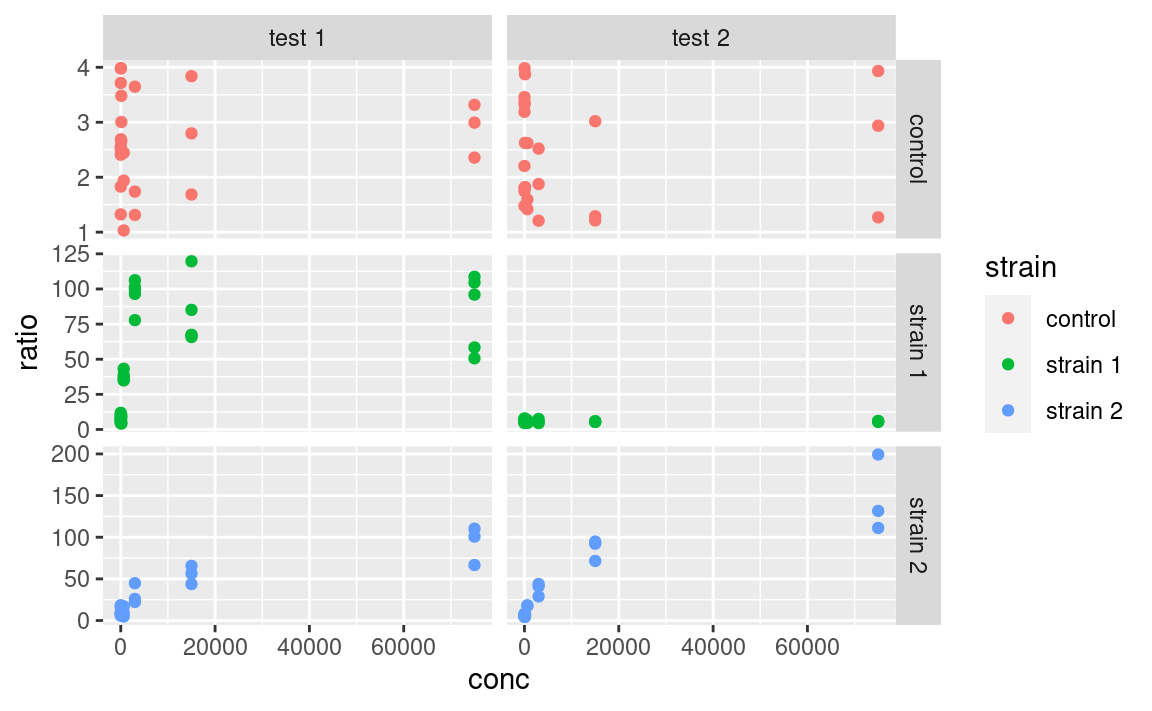`facet_wrap` is similar to `facet_grid`, except that it does not organize the facets in rows and columns but rather as an array of facets that fill the screen by row, like when filling a matrix with numbers:

``````ggplot(data2, aes(x = conc, y = ratio, color = strain)) +
geom_point() +
facet_wrap(strain ~ assay)``````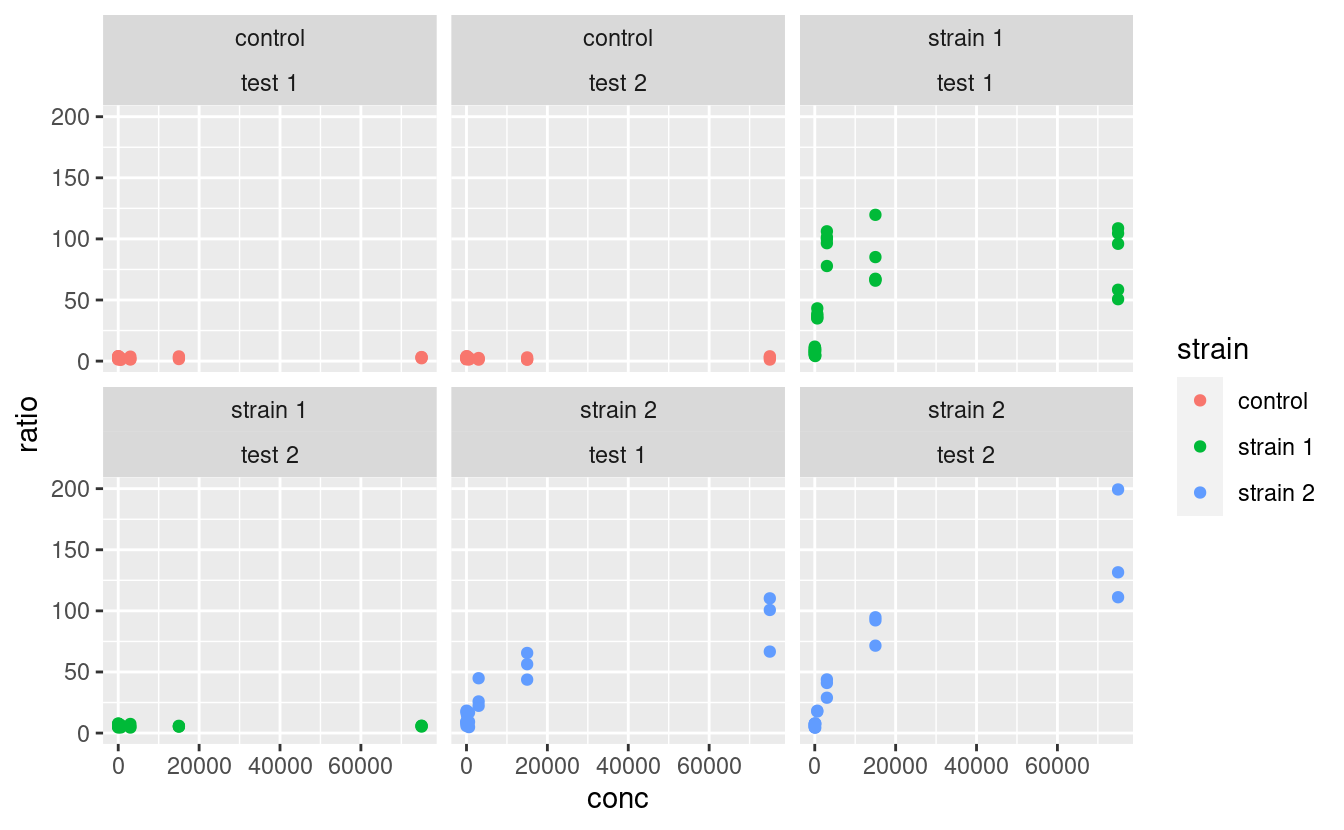where the position of the variables relative to the `~` becomes irrelevant.

Note that a facetted ggplot is still one ggplot, not a combination of ggplots, which we will cover later.

Custom-labelling the strips of the facets is done with the `labeller` argument. The way this is used is a little complicated, but essentially looks like this:

``````ggplot(data2, aes(x = conc, y = ratio, color = strain)) +
geom_point() +
facet_grid(strain ~ assay, labeller = labeller(.rows = label_both))``````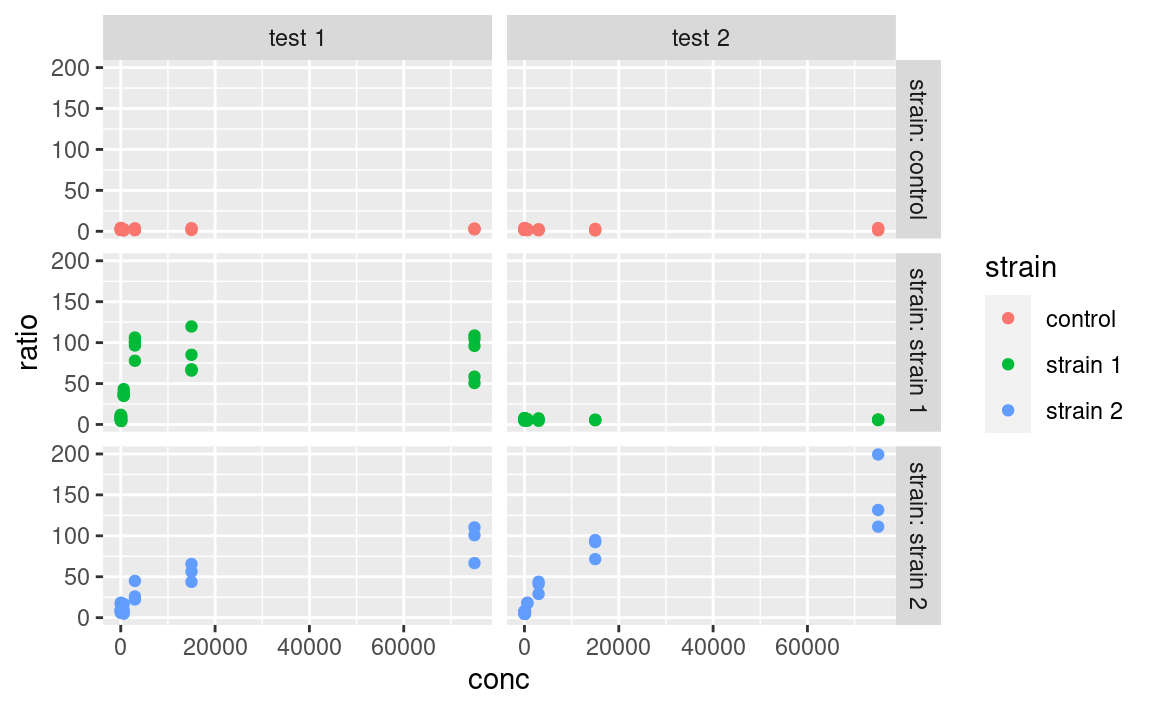Here, the `label_both` function is applied to the variable facetting by row, which is `strain`. `label_both` tells the `labeller` to label the strips with the name of the variable (`strain`) followed by its value, separated by a colon. We will not cover labelling in details here, but keep in mind that the `labeller` argument is what to play with, and that it takes the output of the `labeller` function as input, which itself takes labelling functions, such as `label_both`, as arguments. Other labelling functions include `label_value`, which just shows the value in the strip (that is the default) and `label_parsed`, which is used for showing mathematical expressions in strip labels (e.g. greek letters, exponents etc.). It is possible to provide custom names too. For more information on customizing facet strip lables, visit this link.

Note: I made a package called `ggsim`, yet another extension of `ggplot2` with a few functions coming handy for simulation data. One of the functions, `facettize`, is aimed at making your life easier when labelling the strips of your facets (i.e. not going into the nitty gritty of the `labeller` function), especially when some facets include parsing mathematical expressions. Feel free to install it from GitHub by using:

``devtools::install_github("rscherrer/ggsim")``

## 5.6 The right format for the dataset

One question that may come to your mind is: what is the right format of a dataset for use in `ggplot`, especially since it is part of the tidyverse? The answer is: it depends, and this is where the intergration with other tidyverse tools makes our life easier. If, for example, we want to use a variable for facetting or as an aesthetics, it is important to have this variable as a single column. For example, in the original `data` dataset, we could have compared the optical density between the two time point:

``````ggplot(data, aes(x = time, y = OD600)) +
geom_violin()``````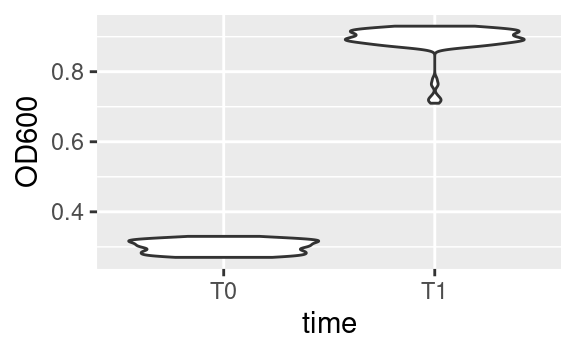where `time` is both an aesthetic (`x`) and its own column. However, if we want to plot the optical density of time point T1 versus that of time point T0, then we need these two time points in separate columns, which is exactly what `OD600_T0` and `OD600_T1`, in the `data2` dataset, are (remember we got those using `tidyr::pivot_wider`):

``````ggplot(data2, aes(x = OD600_T0, y  = OD600_T1)) +
geom_point()``````## 5.7 Plotting as part of a pipeline

What we just saw means that sometimes reformatting of a dataset is needed (e.g. using `pivot_longer` or `pivot_wider` from `tidyr`) to get this one plot done that requires reshaping. If you do not want to spend space storing a reformatted data frame into a whole new object, just to make a single plot, you can use `ggplot` as final part of a tidyverse pipeline. For example, starting from the original data:

``````data %>%
pivot_wider(names_from = "time", values_from = c("cfu", "OD600")) %>%
ggplot(aes(x = OD600_T0, y = OD600_T1)) +
geom_point()``````Notice the use of the pipe `%>%` to pass the resulting data frame on to the `ggplot` command. Because `ggplot` is called with a pipe, its first argument is already passed (it is the data frame coming through the pipe), so we only need to pass the second argument, i.e. the aesthetics mapping, to the `ggplot` function.

## 5.8 Customization

Now that we saw everything there is to know about structuring a `ggplot`, it is time to learn how to polish it (the easiest and most rewarding part!).

### 5.8.1 Scales

Every aesthetics can be scaled. This includes specifying what values an aesthetics can take (e.g. what colors to pick, or what range of transparencies to use), possible break points along the legend, or legend titles and labels, among others. Use the `scale_*` family of functions for that. There are many such functions, because many aesthetics can be modified, but the logic behind their naming is always the same:

``scale_<AESTHETIC>_<TYPE>``

where `<AESTHETIC>` is replaced by the aesthetic you want to scale (e.g. `color`, `size`, `alpha`) and `<TYPE>` is the type of variable that is mapped to this aesthetic (common types are `continuous`, `discrete` and `manual`). Some scaling functions do not take a `<TYPE>` but just an `<AESTHETIC>` in their name, e.g. `scale_alpha`.

In our example, if we color-code points according to their `strain`, which is a categorical variable, we can use `scale_color_manual` (aka `scale_colour_manual`) to manually pick the colors we want:

``````ggplot(data2, aes(x = conc, y = ratio, color = strain)) +
geom_point() +
geom_smooth() + # just to spice up our use of geoms
facet_grid(strain ~ assay) +
scale_color_manual(values = c("forestgreen", "goldenrod", "mediumseagreen"))``````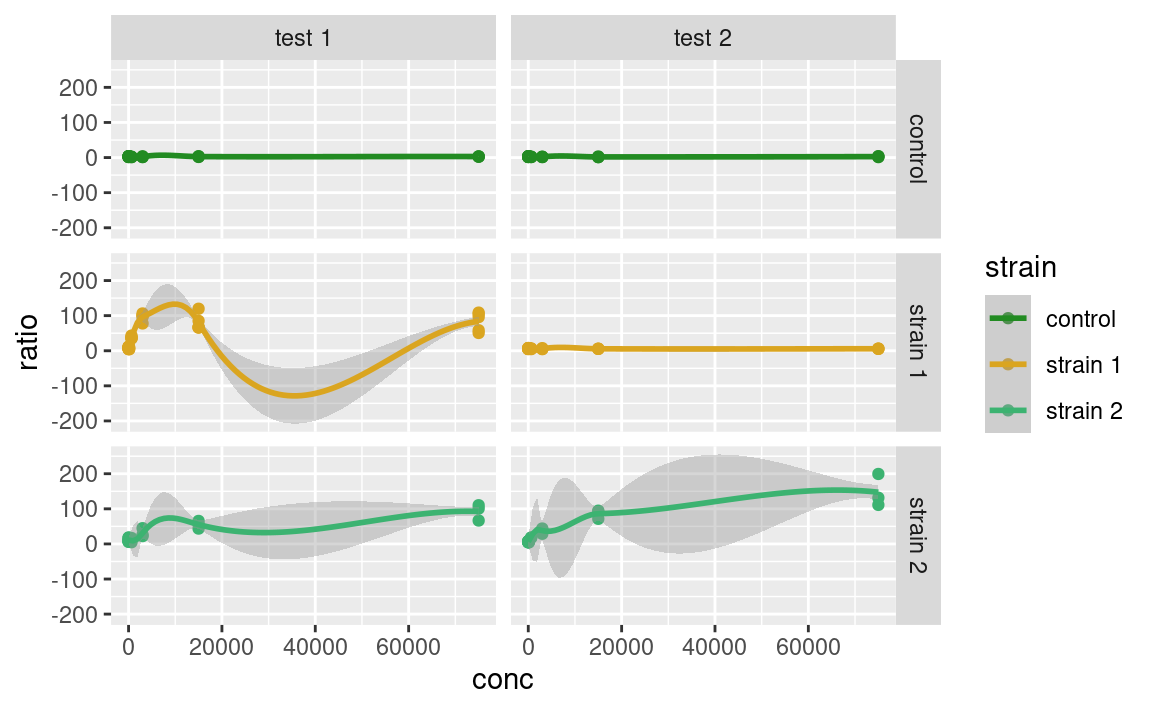Alternatively, we could color-code the points based on their number of CFU at time point T1, `cfu_T1`, which is a continuous variable, using `scale_color_continuous`. Without scaling:

``````ggplot(data2, aes(x = conc, y = ratio, color = cfu_T1)) +
geom_point() +
facet_grid(strain ~ assay)``````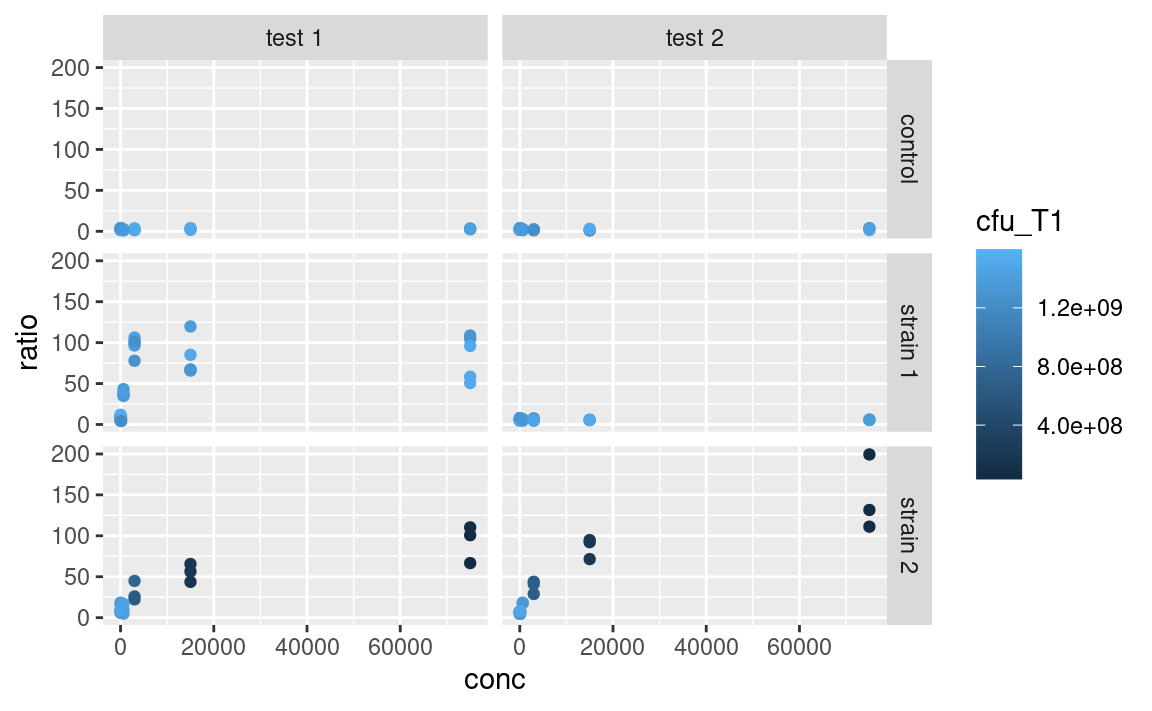With scaling:

``````ggplot(data2, aes(x = conc, y = ratio, color = cfu_T1)) +
geom_point() +
facet_grid(strain ~ assay) +
scale_color_continuous(type = "viridis")``````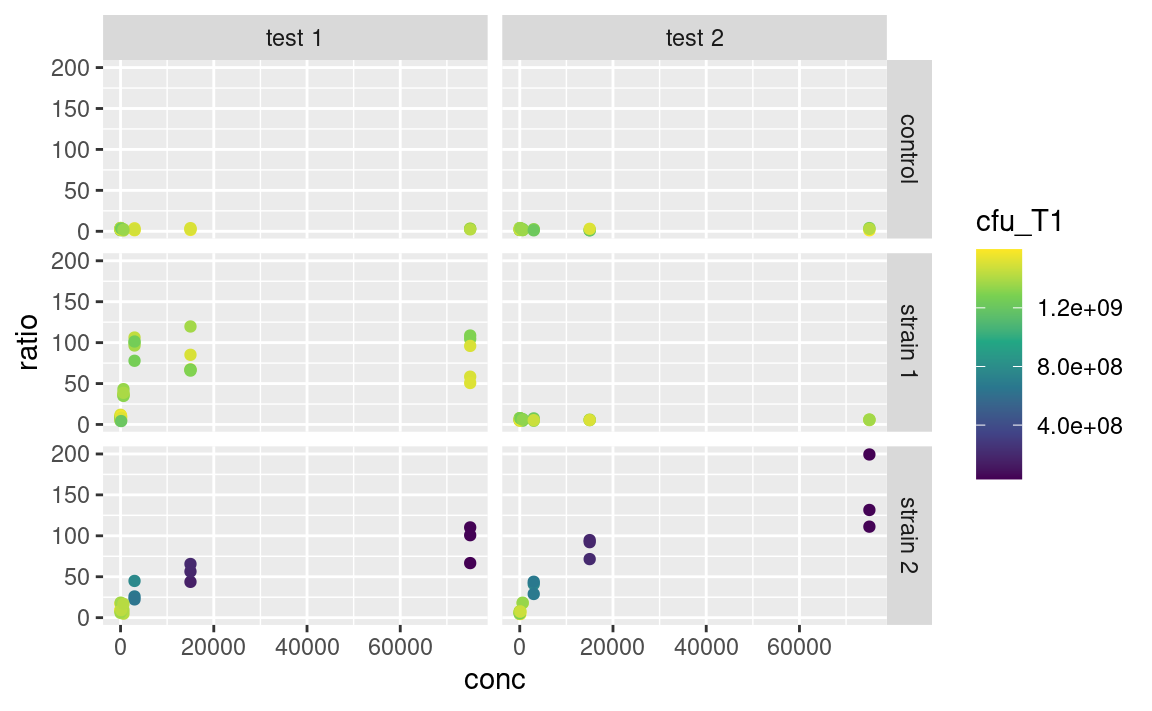The arguments that are taken by the `scale_` function really depend on the use case, e.g. `scale_color_manual` expects discrete `values`, `scale_color_continuous` expects a `type` of built-in continuous color gradient, and `scale_color_gradient` expects a `low` and `high` color boundaries (and also a `mid`-gradient color in the case of `scale_color_gradient2`). But the logic shown here is similar across many aesthetics, e.g. `scale_alpha_continuous` and `scale_size_continuous` work in similar ways, both taking a `range` argument. So, lots of scaling functions to play with, of which we do not provide an exhaustive list here.

Mandatory aesthetics, such as `x` and `y`, also have their scaling functions. If `x` or `y` is continuous, one can e.g. use `scale_x_log10` to show this axis on a logarithmic scale, without having to log-tansform the data before plotting, e.g.

``````ggplot(data2, aes(x = conc, y = ratio, color = cfu_T1)) +
geom_point() +
facet_grid(strain ~ assay) +
scale_color_continuous(type = "viridis") +
scale_x_log10()``````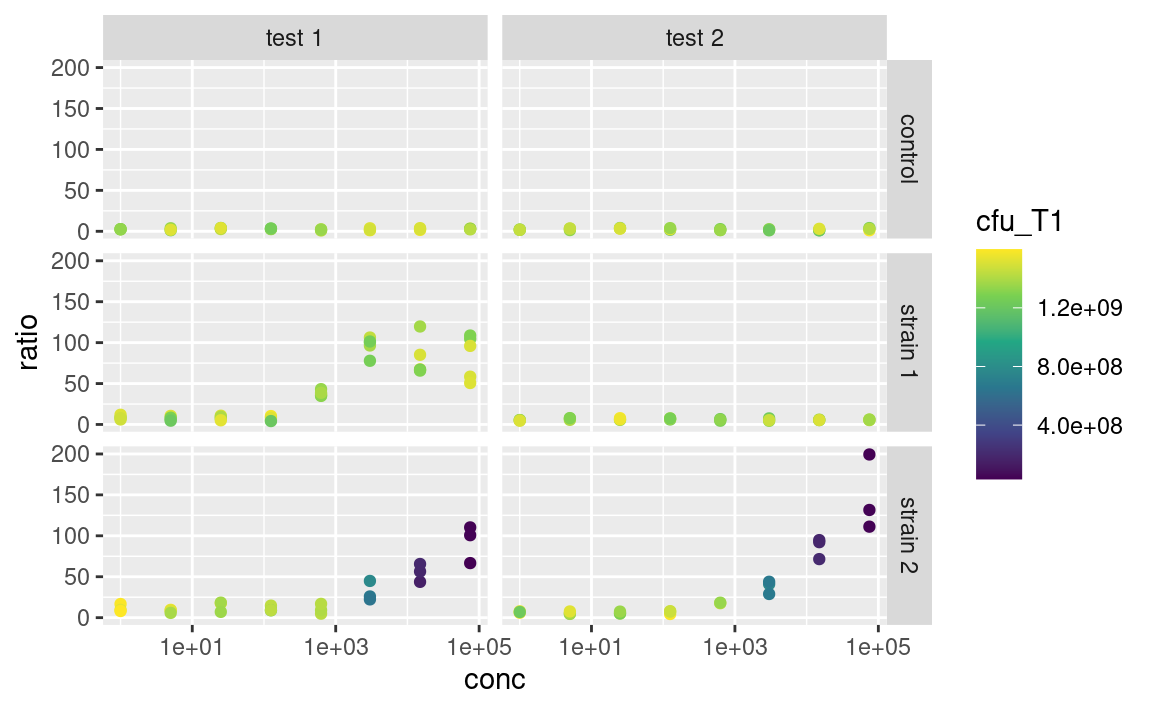More on re-scaling legend titles and labels further down.

### 5.8.2 Labels

The functions `ggtitle`, `xlab`, `ylab` and `labs` allow you to customize the labels shown for each aesthetics (remember that the x- and y-axes are aesthetics too), and for the main title of the plot. On to a full-fledge example:

``````p <- ggplot(data2, aes(x = conc, y = ratio, color = cfu_T1)) +
geom_point() +
facet_grid(strain ~ assay) +
scale_color_continuous(type = "viridis") +
scale_x_log10() +
xlab("Nisin concentration (mmol/mL)") +
ylab("Mutational ratio") +
labs(color = parse(text = "'CFU at '~T")) + # plotmath expression
ggtitle(
"A very important experiment",
"So important it deserves a subtitle"
)
p``````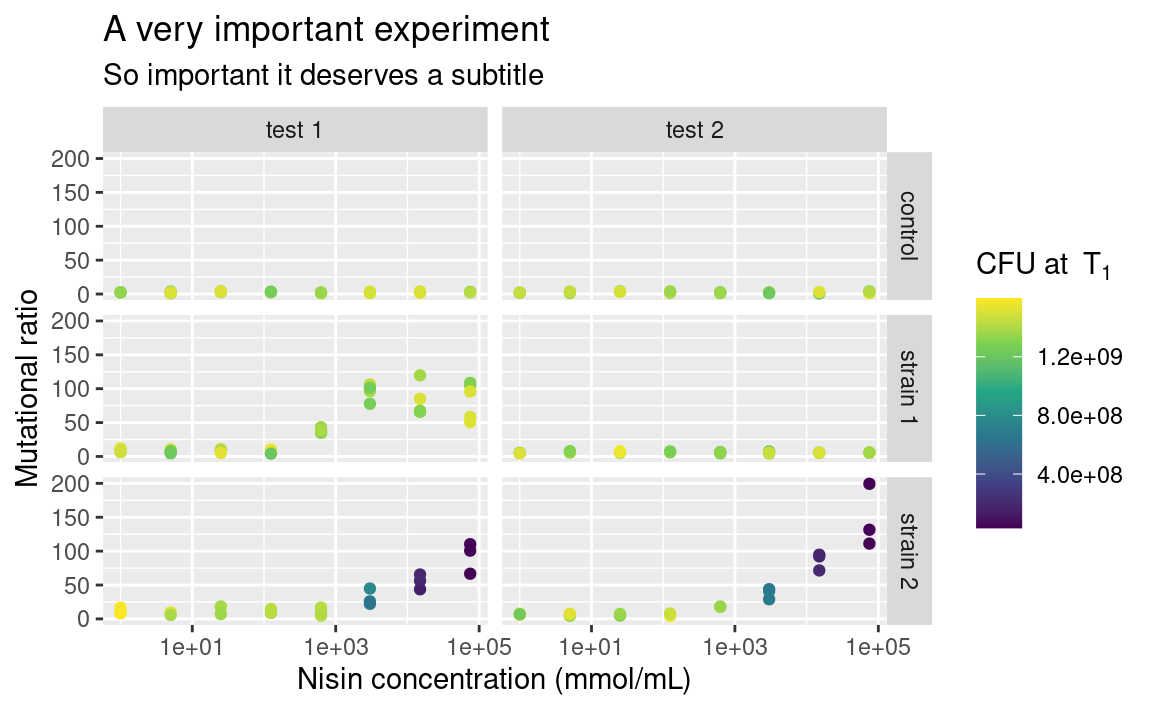Note that `xlab` and `ylab` are wrappers around `labs`, meaning that we could have provided `labs` with `x = ...` and `y = ...` in addition to `color = ...`, its arguments just need to take the names of the aesthetics. If you want no labels, use e.g. `xlab(NULL)` or `ylab(NULL)`.

Also notice the use of `parse` to display mathemetical notations using the `plotmath` syntax. This is not part of the tidyverse though, so it is a story for another day, feel free to look it up (type `?bquote`)!

### 5.8.3 Themes

You may be already frustrated that all plots have this same grey default `ggplot2` background. Of course, it is possible to change this too by playing with the `theme` functions. There are other built-in themes than the default grey one, such as `theme_bw` or `theme_classic`:

``````p + theme_classic()
p + theme_bw()``````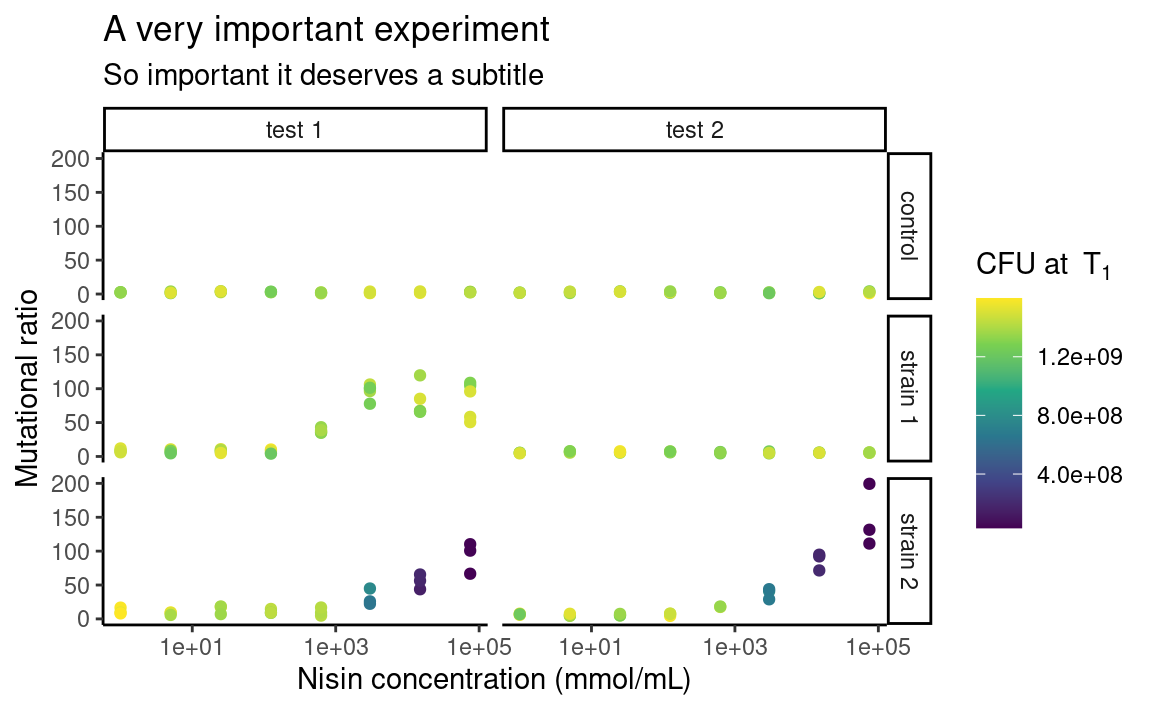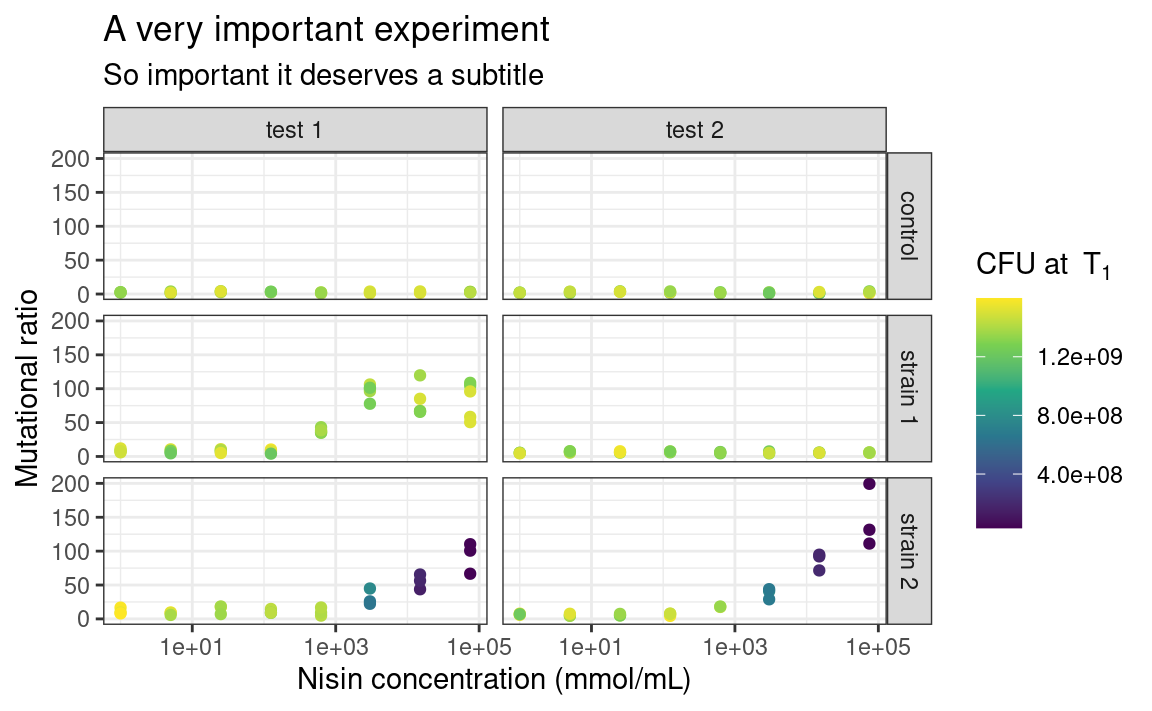The individual elements of the theme, e.g. the background grid or the color of the panel, can be customized using the arguments in the `theme` function. The `theme` function can also be used to modify stuff related to the legend or the axes of the plots. For example:

``````p <- p +
theme_bw() +
theme(
legend.position = "left",
axis.text.x = element_text(angle = 60, hjust = 1)
)
p``````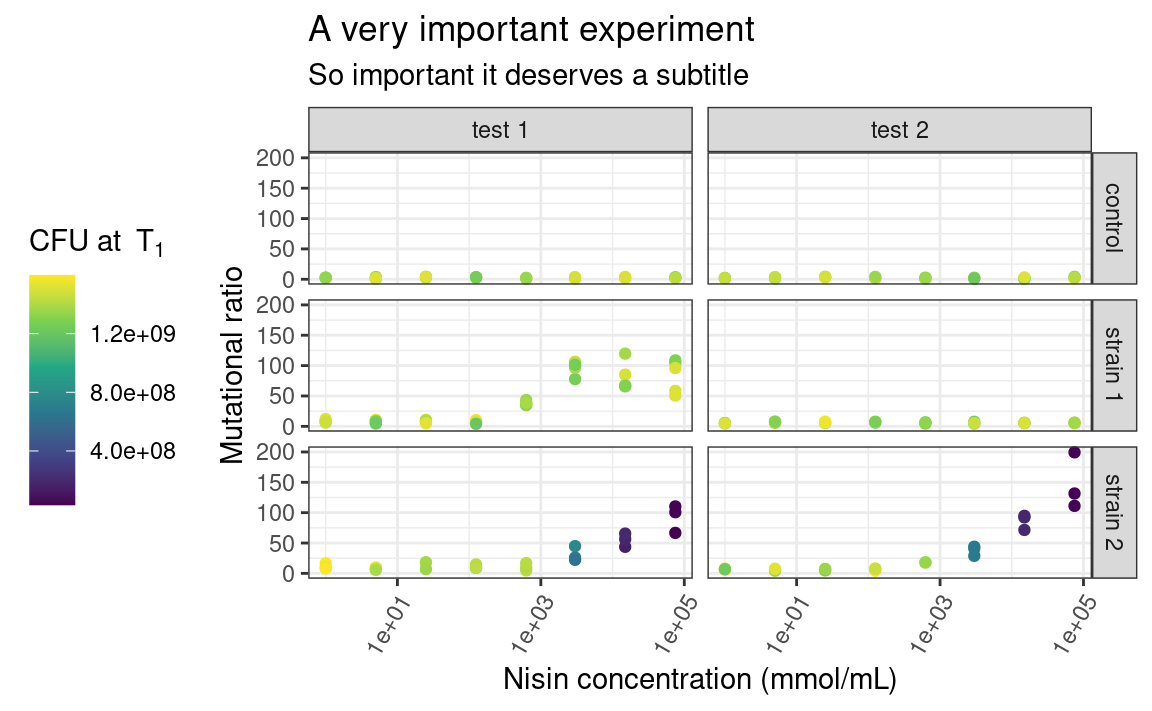Here, `legend.position` is sort of self-explanatory, but `axis.text.x` is a bit more subtle. Some elements of the theme, such as the text of the axes, need a series of graphical parameters in order to be modified, and the graphical parameters that can be used depend on the type of object those theme elements are (are they `text`, `rect` or `line`?). We use the `element_*` family of functions to pass those graphical parameters to our theme elements of interest. Here, we use `element_text` to transform the `text` on the x-axis by rotating it by an `angle` of 60 degrees, and then align each label to the right (`hjust` stands for “horizontal justification”). Again, lots of combinations are possible. Explore!

### 5.8.4 Legend

The one thing I Google the most, without a doubt, is “custom legend in ggplot”, because I always forget how to choose which legend to show, e.g. if I want to display the color legend but not the alpha legend. So here it is: to hide all the legends, use:

``p + theme(legend.position = "none")``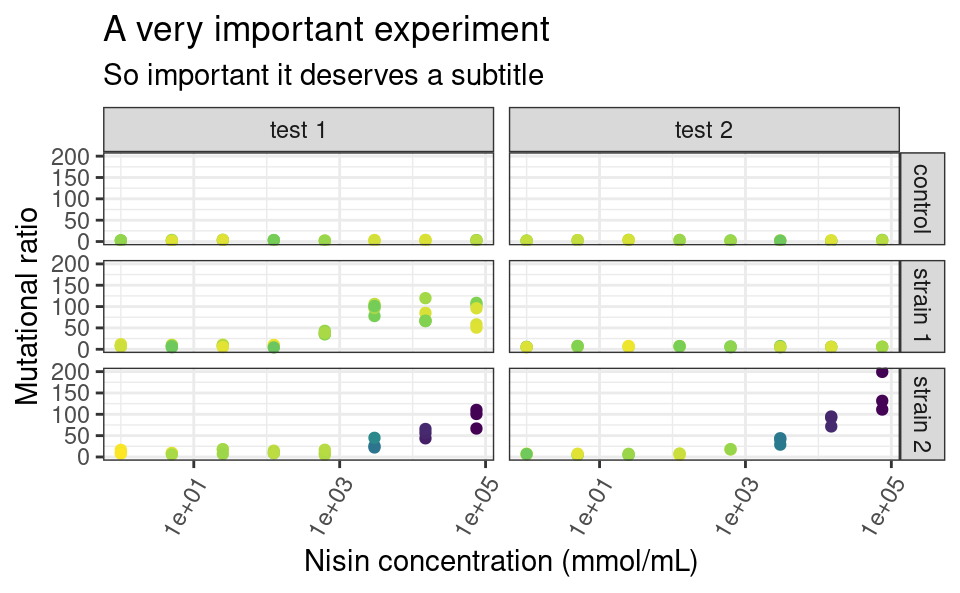And to selectively hide some legends, use `guides`:

``p + guides(color = FALSE)``It is also important to remember that `ggplot2` will try to combine legends together whenever it can. If the same variable is mapped to two different aesthetics, e.g. shape and color, only one legend will appear:

``````ggplot(data2, aes(x = conc, y = ratio, color = strain, shape = strain)) +
geom_point() +
facet_grid(strain ~ assay) +
scale_x_log10()``````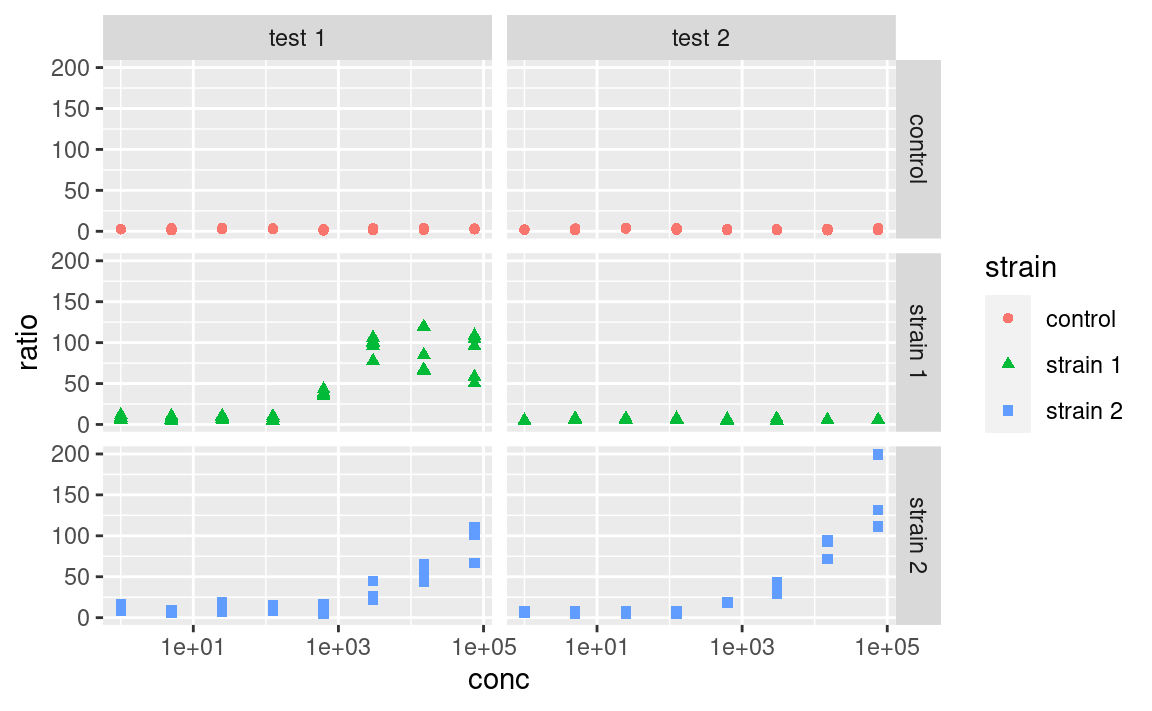But this behavior can be controlled. You can use the arguments of the `scale_` functions to pass custom titles and labels to the legends. And if the legends mapping to the same variable have different titles or labels, they will be shown separately:

``````ggplot(data2, aes(x = conc, y = ratio, color = strain, shape = strain)) +
geom_point() +
facet_grid(strain ~ assay) +
scale_x_log10() +
scale_color_manual(
"color legend", values = c("forestgreen", "goldenrod", "mediumseagreen")
) +
scale_shape_manual(
"shape legend", values = c(16, 17, 18),
labels = c("Control", "Strain 1", "Strain 2")
)``````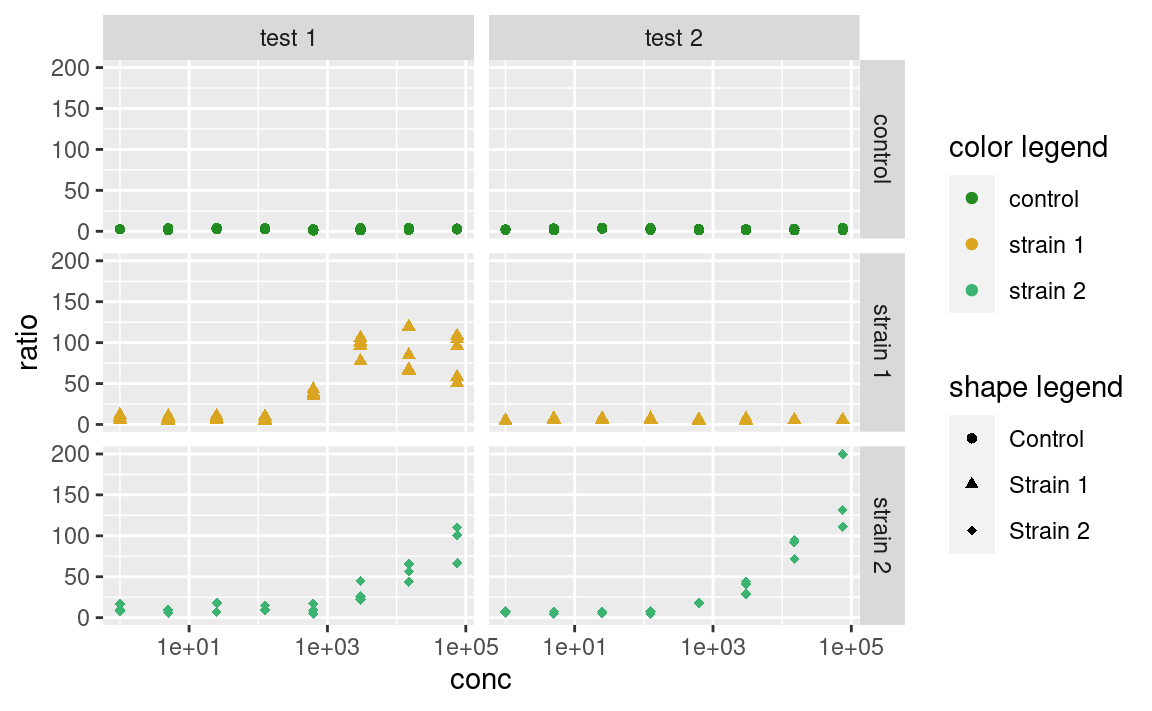Note that you can also use this trick to combine different legends together, by giving them the same titles and labels.

## 5.9 Combining plots

This was more or less what you need to know to be operational when plotting single ggplots. But what if the facetting option is not enough, and you want to combine multiple plots into a single figure? `ggplot2` itself does not do that, but the good news is, there are many packages that do. Those include `patchwork`, `cowplot`, `grid`, `gridExtra`, `egg` or `aplot` (and probably more).

One term that these packages often use is `grob`. A `grob` is a ggplot-like object, such as a `ggplot` but could also be a single text label in the middle of a plotting window. These packages essentially assemble grobs together.

`patchwork` is personally my favorite so I will focus on this one here. It has the advantage to automatically align the frames of the different plots across the different subplots (I found that this is not entirely true when combining `ggtree` objects with other plots, `aplot` is better for this specific case). It also has an excellent, succinct documentation.

Let us look at an example, where we assign the previous plot to `p1` and make a new plot to combine it with, called `p2`:

``````p1 <- p
p2 <- ggplot(data2, aes(x = strain, y = OD600_T1, color = strain)) +
geom_violin(draw_quantiles = 0.5) +
geom_jitter(width = 0.2) +
theme_classic() +
xlab(NULL) +
ylab(parse(text = "'Optial density at 600nm at'~T")) +
theme(legend.position = "none")
p2``````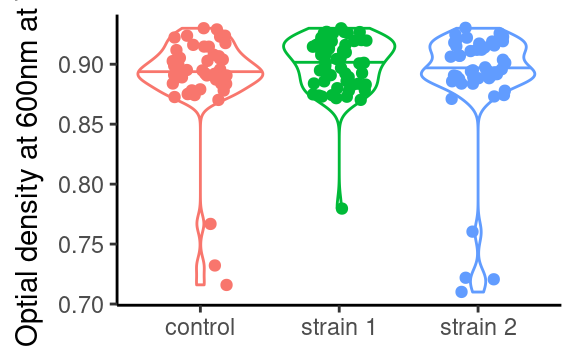In `patchwork`, we would combine both using:

``````library(patchwork)
p1 + p2``````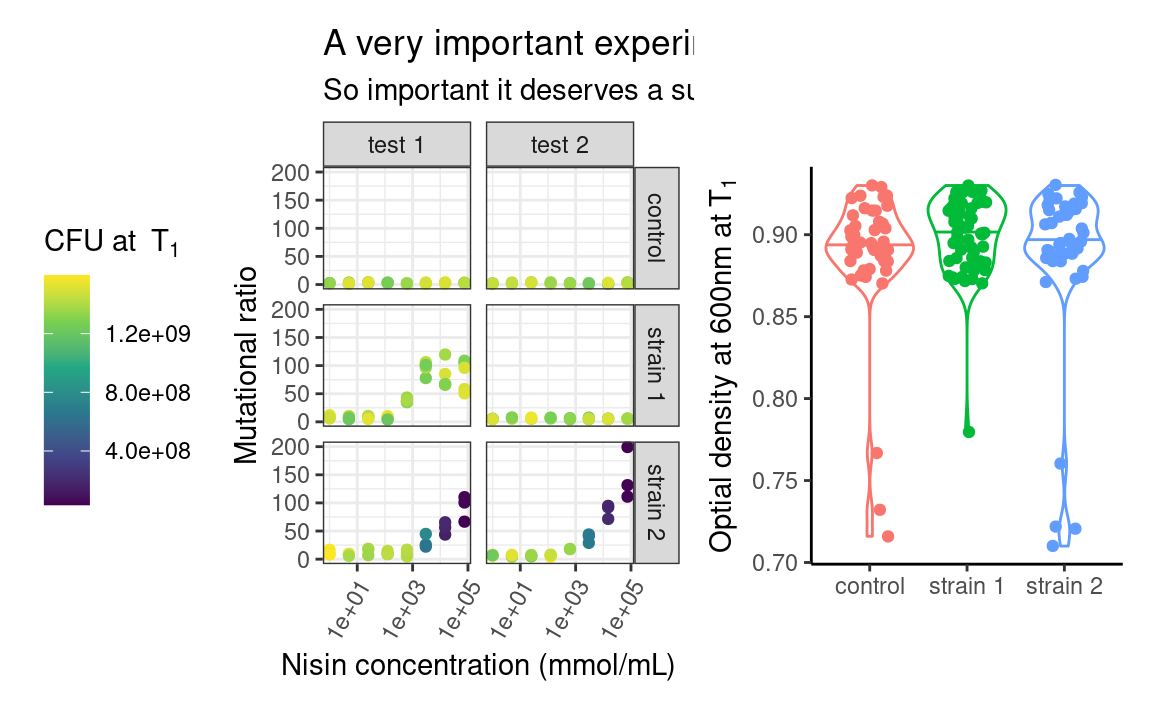`patchwork` uses operators such as `+`, `/` or `|` to assemble the plots in various layouts. It looks simple, but a caveat of this approach is that it may become tedious when assembling, e.g. 15 small plots, or plots from a list of unknown length. The programmatic equivalent of the above example is:

``wrap_plots(p1, p2) # or even more programmatic, wrap_plots(list(p1, p2))``More customization can be added to the previous combination of plots, such as layout specifications, e.g. controlling the position and dimension of the different plots, or annotations, e.g. global title, labelling each plot or capturing the legends of all the plots and show it as one global legend). But this is a `ggplot2` tutorial and we just want you to know that `patchwork` and friends exist, so go check them out to know more about what they can do!

## 5.10 Saving a plot

Last but not least, ggplots have their own saving function: `ggsave` (it also works on combinations of ggplots made by `patchwork` or `cowplot`), which guesses the extension of your figure (e.g. `.png` or `.pdf`) from the file name you provide. You can also give it specific `width`, `height` and `dpi` (resolution) parameter values.

## 5.11 High throughput plotting workflow

As we mentioned in the part about combining plots, sometimes we want to do things many times (in my case I often make 100 times the same figure, just for different replicate simulations). Of course we would not copy and paste many times the same snippet of code, or write 100 times `+` to assemble some plots (by now we are advanced R users, after all). This is where we can make use, again, of the combination of tidyverse tools, and especially `purrr`.

Let us make a function that plots the number of CFU against the optical density, facetted by time point (so, that function expects a time point-wise dataset, such as `data`):

``````plot_this <- function(data) {
ggplot(data, aes(x = OD600, y = cfu, color = cfu)) +
geom_point() +
facet_grid(. ~ time) +
theme_classic() +
scale_color_continuous(type = "viridis") +
theme(legend.position = "none") +
xlab(parse(text = "'OD at 600nm at'~T")) +
ylab("CFU")
}``````

Note that this does not plot anything, it is just a function that will if called on a dataset.

The objective is to apply this function to each `strain`-`assay` combination, thus getting one plot per combination. We can check that this function works as expected for a single combination using our friend `dplyr`:

``````data %>%
filter(strain == "control", assay == "test 1") %>%
plot_this()``````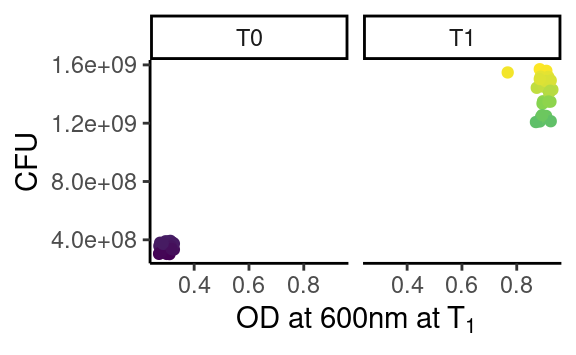which works because `plot_this` takes a data frame as first argument.

Now that we are happy with out single-plot function, we `tidyr::nest` our data frame into all the relevant combinations of `strain` and `assay`, and we `purrr::map` through the resulting list-column to produce many ggplots in one go:

``````newdata <- data %>%
group_by(assay, strain) %>%
nest() %>%
mutate(fig = map(data, plot_this))
newdata
#> # A tibble: 6 x 4
#> # Groups:   strain, assay 
#>   strain   assay  data              fig
#>   <chr>    <chr>  <list>            <list>
#> 1 strain 1 test 1 <tibble [72 × 5]> <gg>
#> 2 control  test 1 <tibble [48 × 5]> <gg>
#> 3 strain 2 test 1 <tibble [48 × 5]> <gg>
#> 4 strain 2 test 2 <tibble [46 × 5]> <gg>
#> 5 strain 1 test 2 <tibble [48 × 5]> <gg>
#> 6 control  test 2 <tibble [48 × 5]> <gg>``````

where the new list-column `fig` is a list of `ggplot` objects, that we can check individually:

``newdata\$fig[]``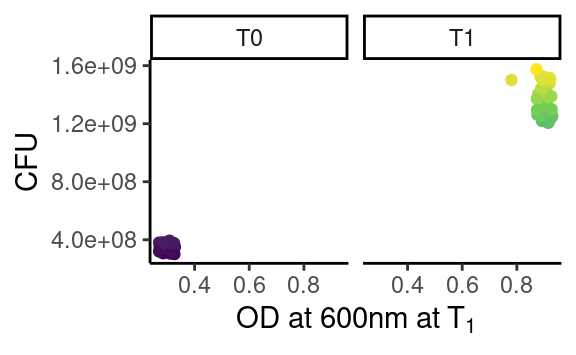Looks `purrr`fect.

If you ask yourself why going through this hassle whith only two assays and three strains, just think about a case where you would have hundreds of e.g. simulations, sequences, field sites or study species.

Let us go a bit further. Now we want to combine plots for each `strain` into one figure per `assay`. We also want to give the resulting combined plot a figure file name, and save all the figures. There we go:

``````newdata <- newdata %>%
select(-data) %>% # just to clean up a bit
group_by(assay) %>%
nest() %>%
mutate(combifig = map(data, ~ wrap_plots(.x\$fig)))
newdata
#> # A tibble: 2 x 3
#> # Groups:   assay 
#>   assay  data             combifig
#>   <chr>  <list>           <list>
#> 1 test 1 <tibble [3 × 2]> <patchwrk>
#> 2 test 2 <tibble [3 × 2]> <patchwrk>``````

Note that we use the formula-way of passing functions to `map` (using `~`), which is more succinct than the lambda way (using an anonymous function `function(x) wrap_plots(x)`), and where `.x` is interpreted as an element of the list we iterate through (here the list-column `data`). Please refer to the `purrr` documentation for more details.

As we can see, we have created a new list-column `combifig`, filled with `patchwork` objects, i.e. combined plots:

``newdata\$combifig[]``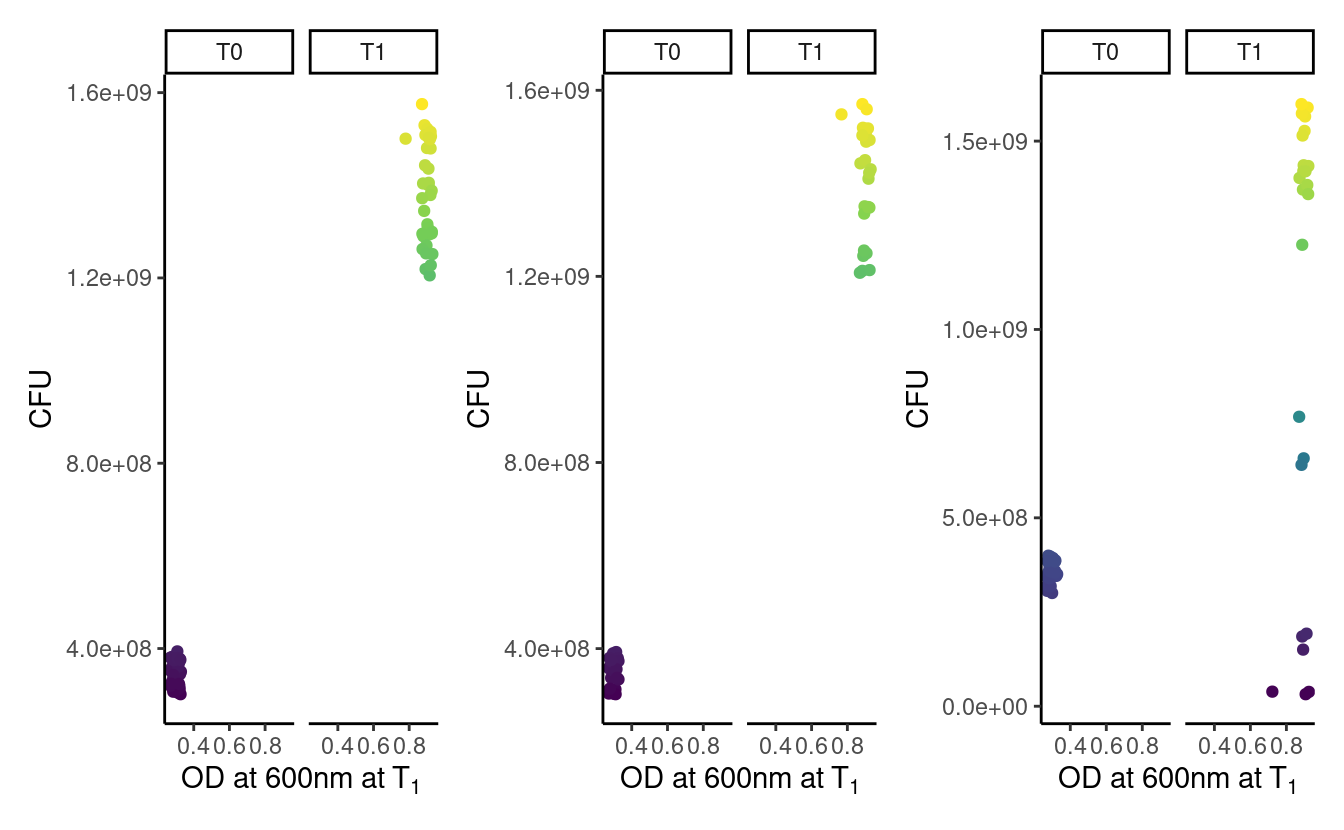We could of course further customize the assembly of plots, but we refer the reader to the `patchwork` documentation for this.

Last step, preparing file names and saving the figures, using old friends from the tidyverse:

``````library(glue)
newdata %>%
mutate(figname = glue("data/figure_{str_replace(assay, ' ', '_')}.png")) %>%
mutate(saved = walk2(figname, combifig, ggsave))
#> # A tibble: 2 x 5
#> # Groups:   assay 
#>   assay  data             combifig   figname               saved
#>   <chr>  <list>           <list>     <glue>                <glue>
#> 1 test 1 <tibble [3 × 2]> <patchwrk> data/figure_test_1.p… data/figure_test_1.p…
#> 2 test 2 <tibble [3 × 2]> <patchwrk> data/figure_test_2.p… data/figure_test_2.p…``````

## 5.12 Want more?

`ggplot2` is undoubtedly one of the largest chunks of the tidyverse. Here we tried to provide a global understanding of how it works, but we could not dig into all possible functions it has (this would take us days). Hopefully now you are armed with the necessary knowledge to be able to find the missing pieces you need.

Some things, however, are missing from `ggplot2`. Fortunately, there are many of extensions building on `ggplot2` that respect the same grammar. Some of them implement new geoms (e.g. such as `ggridges` for ridge-density plots, `ggradar` for radial plots, or `gghalves` for mixes of geoms), others combine plots together (examples cited above), offer more complex themes (e.g. `ggnewscale` for multiple scales of the same type to coexist, or `ggdark` for a dark background), deal with complicated objects that are not trivial to fit in data frames (e.g. `ggtree` for tree-like objects or `ggraph` for networks), or provide shortcuts to quickly produce publication-ready figures for common plot layouts and their corresponding statistical analyses (e.g. `ggpubr`, `ggrapid` or `GGally`). There are even packages for animated graphics (`gganimate`), interactive plot building (`esquisse`) or 3D surface plotting (`rayshader`). See the links below!